### Solving the Megaminx Last Layer with Algorithms

In this guide we're going to explain how to solve the last layer of the Megaminx puzzle with a full list of algorithms. Simply match the last layer state of your Megaminx with one of the images below and use the corresponding algorithm to move on to the next step. This is known as 4-look last layer.

Download our full Megaminx Last Layer Algorithms PDF to take these algs with you on the go

### Megaminx Last Layer - Edge Orientation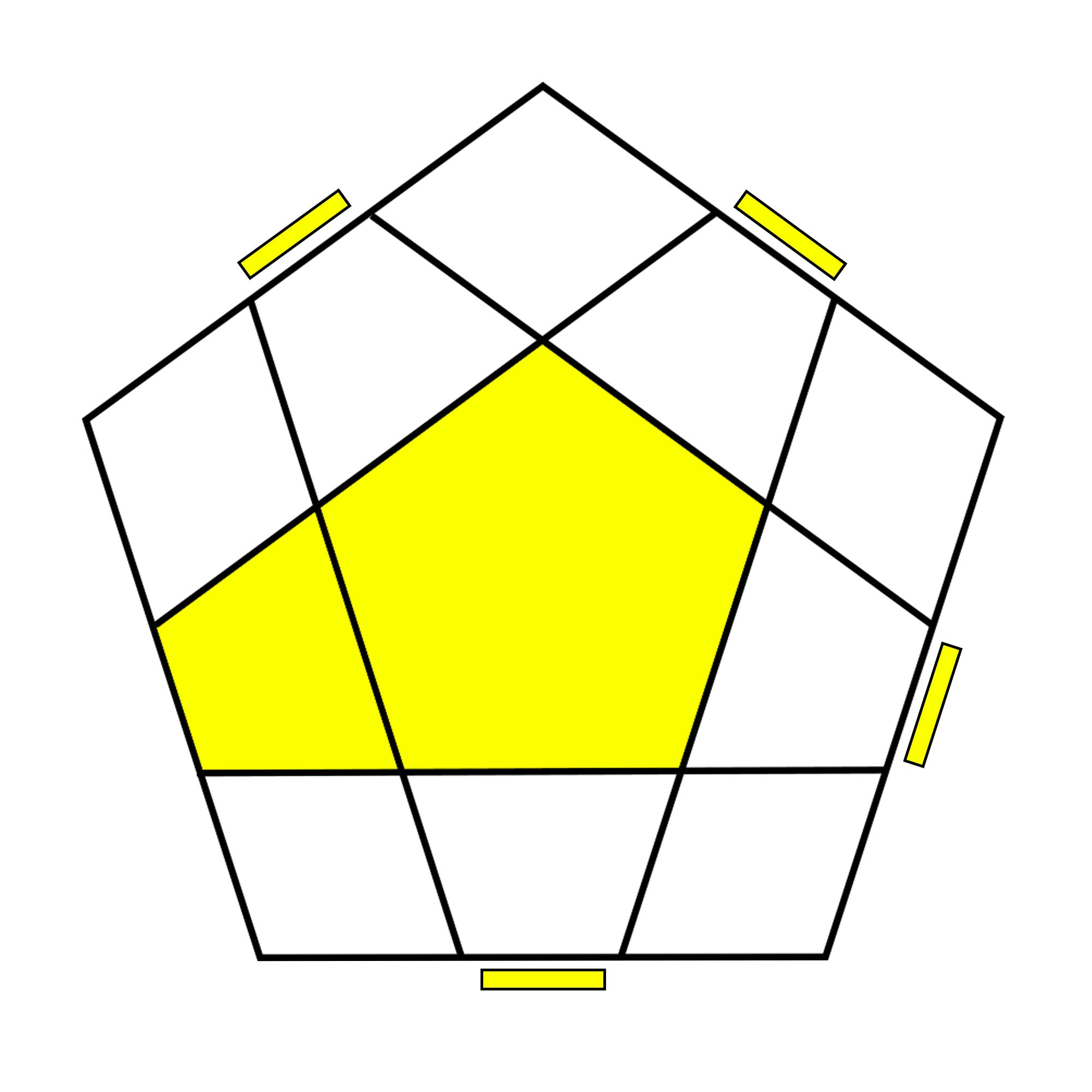F R U2 (R2' F R F') U2' F'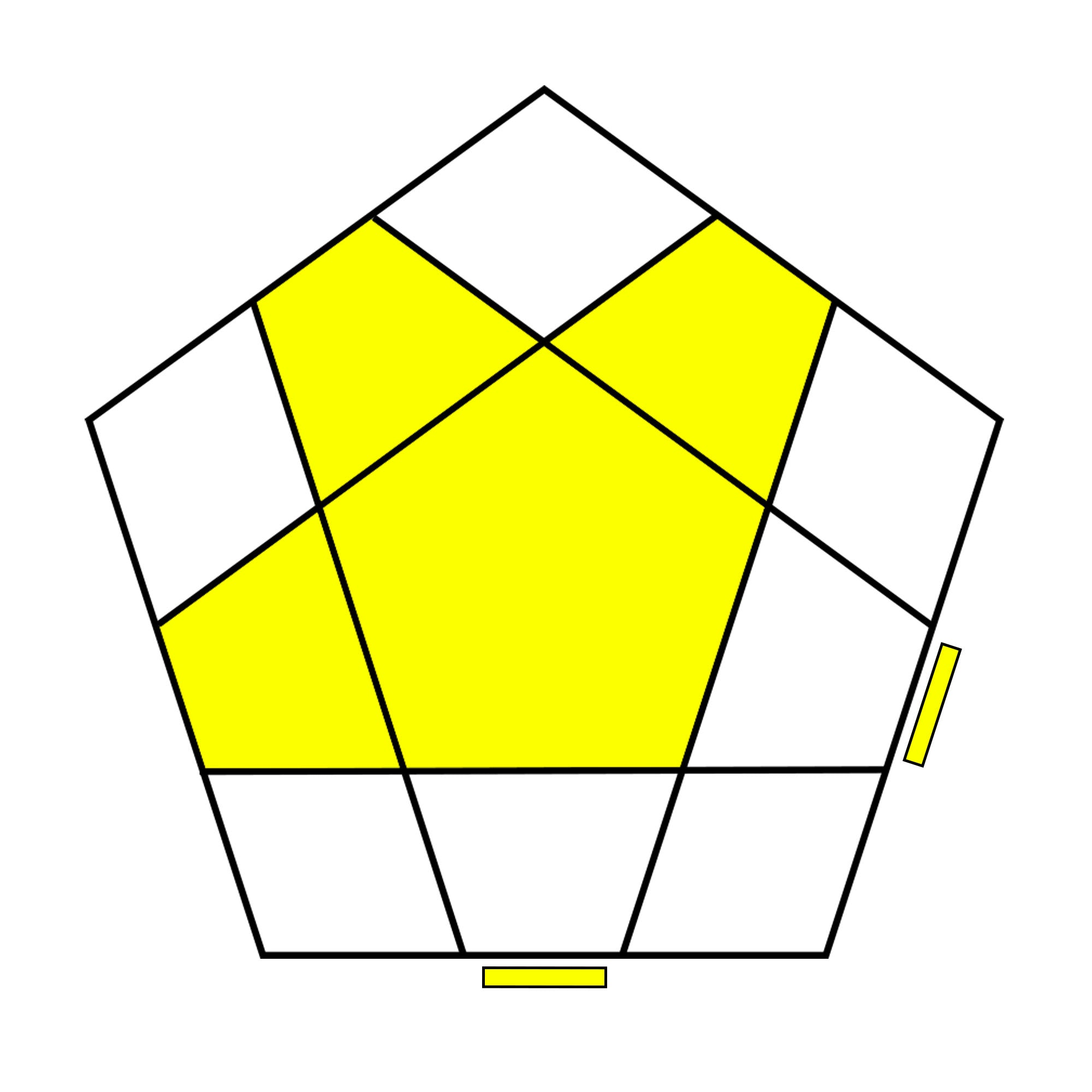F (U R U' R') F'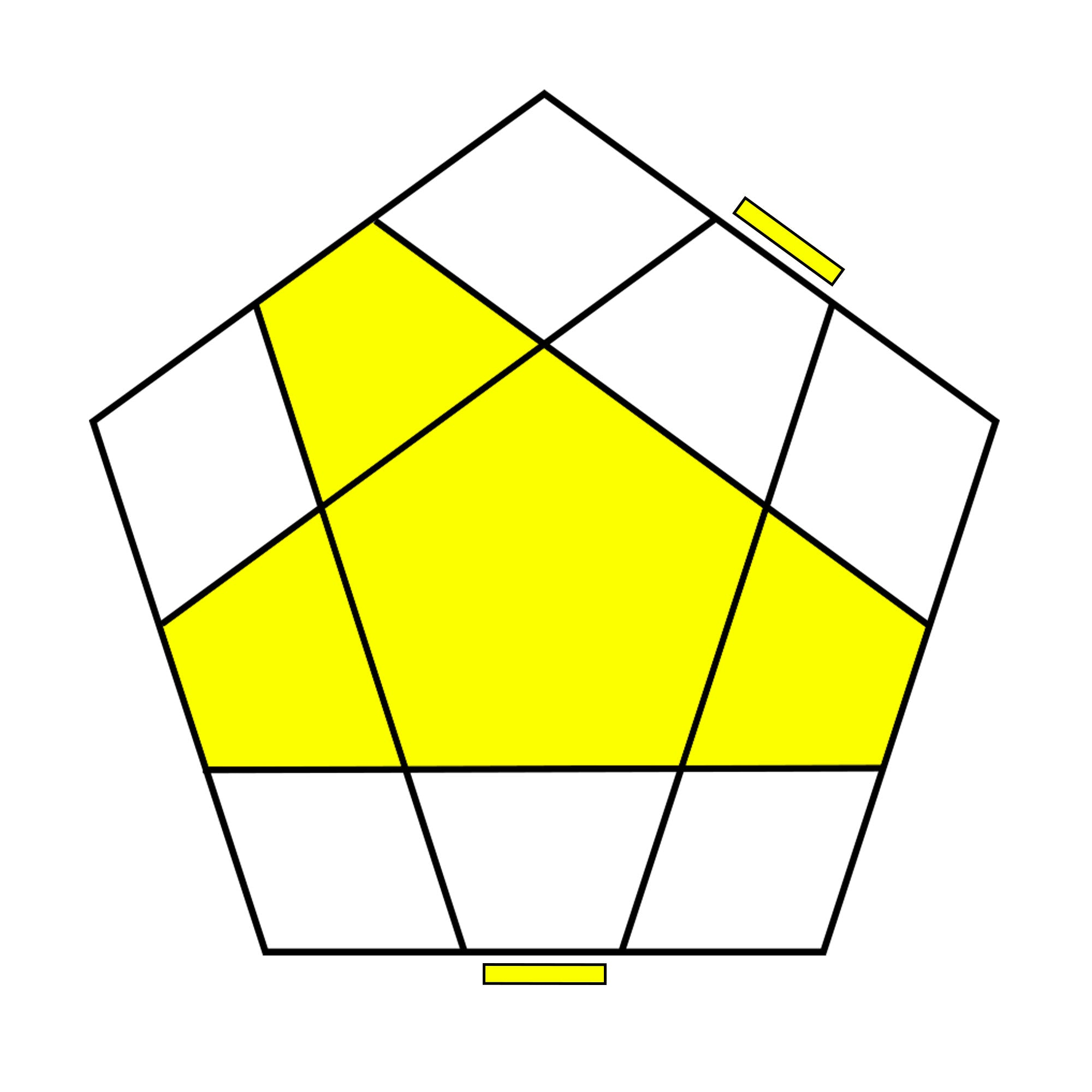F (R U R' U') F'

### Megaminx Last Layer - Corner Orientation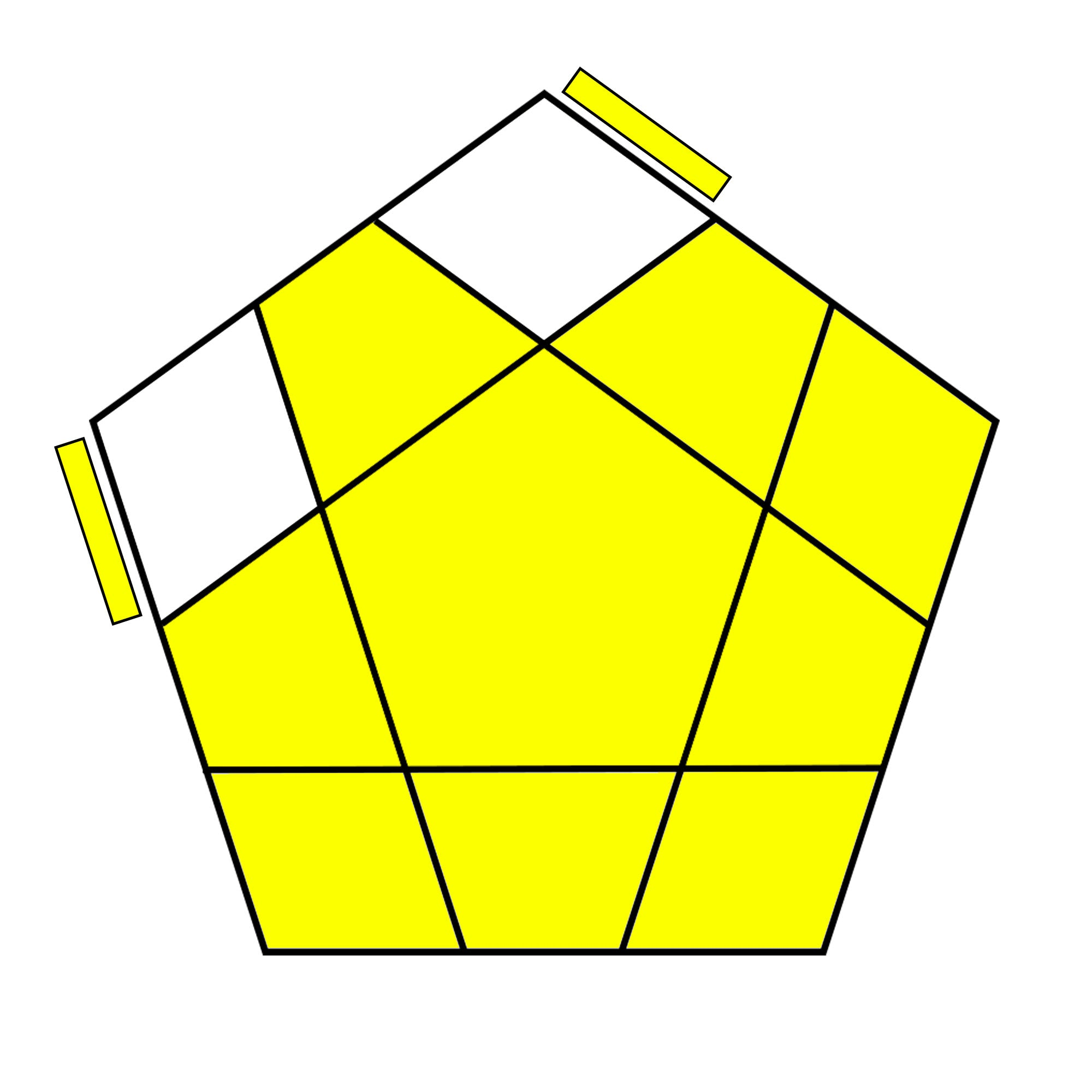F (R U2 R' U' R U' R') F'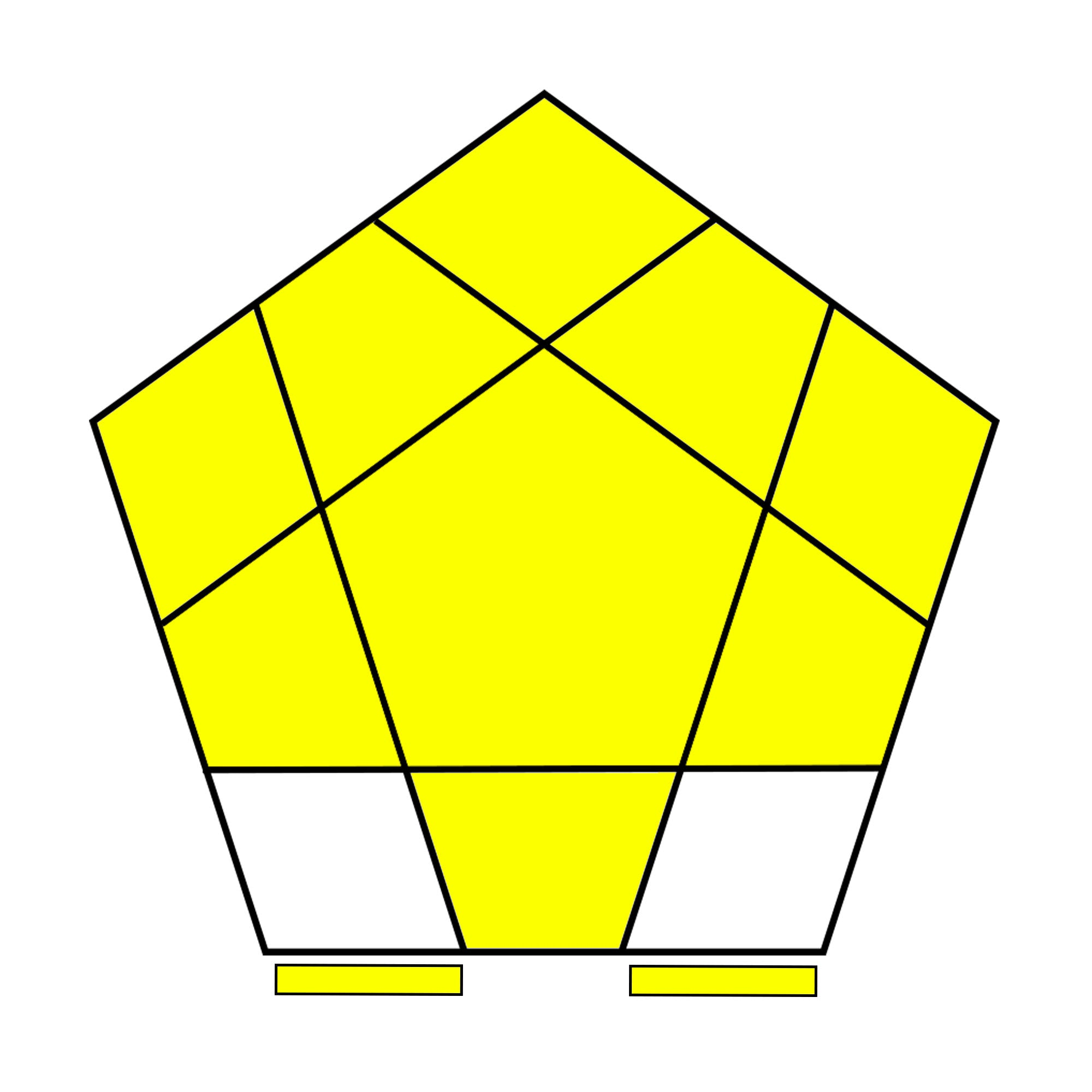(R U R' U) (R U R' U2') (R U' R')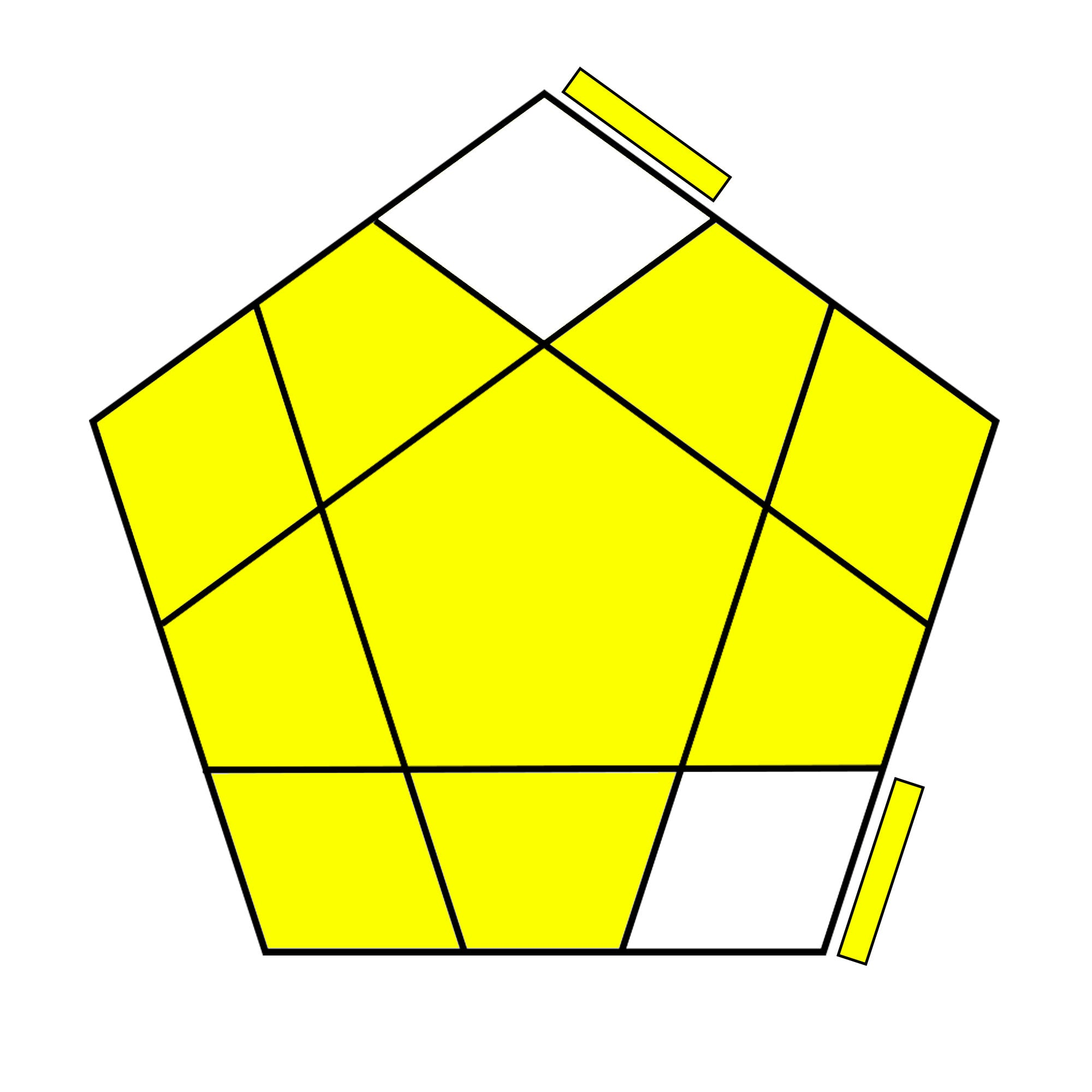(R U R' U') (R' F R U) (R U' R' F')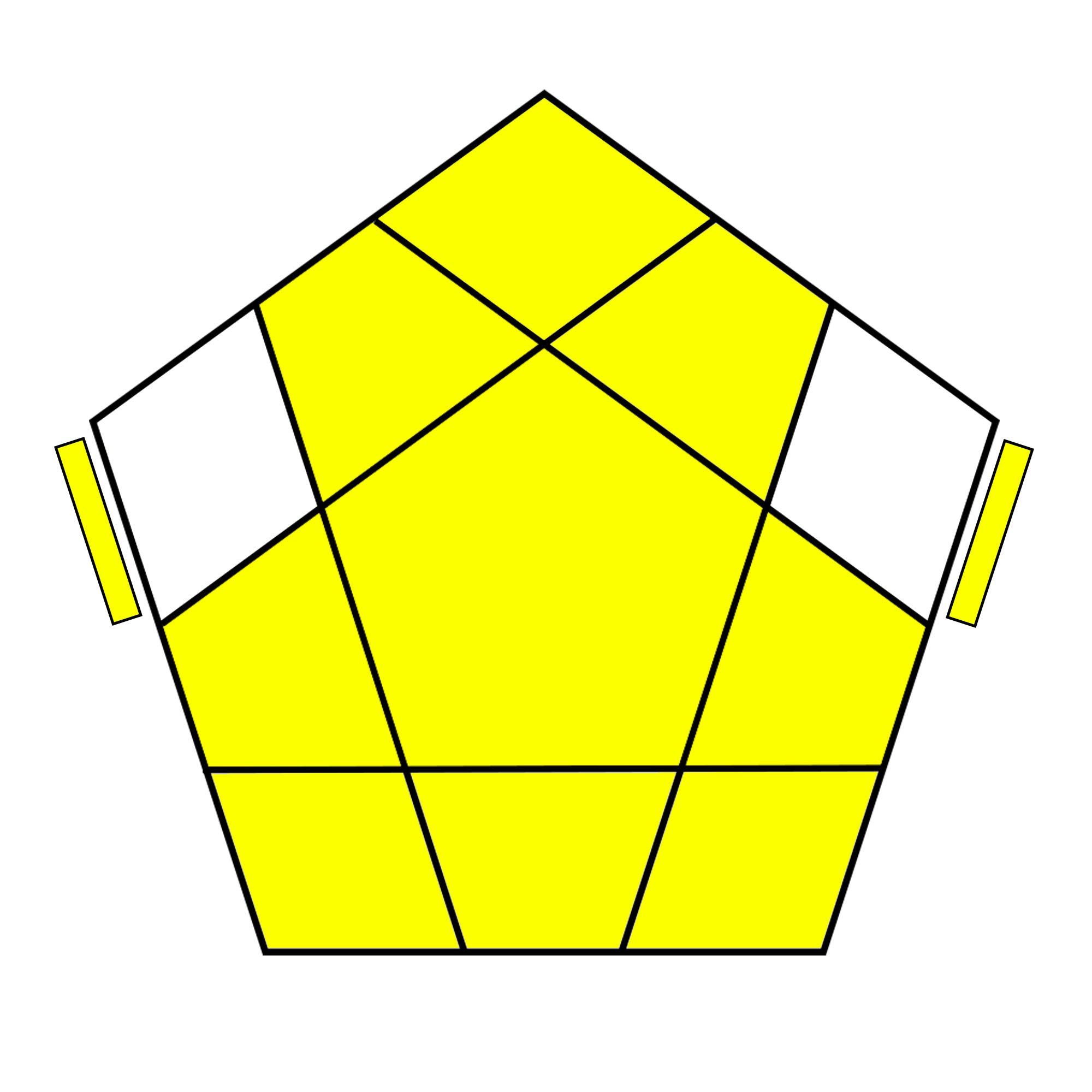(R U2 R') U (R U2 R')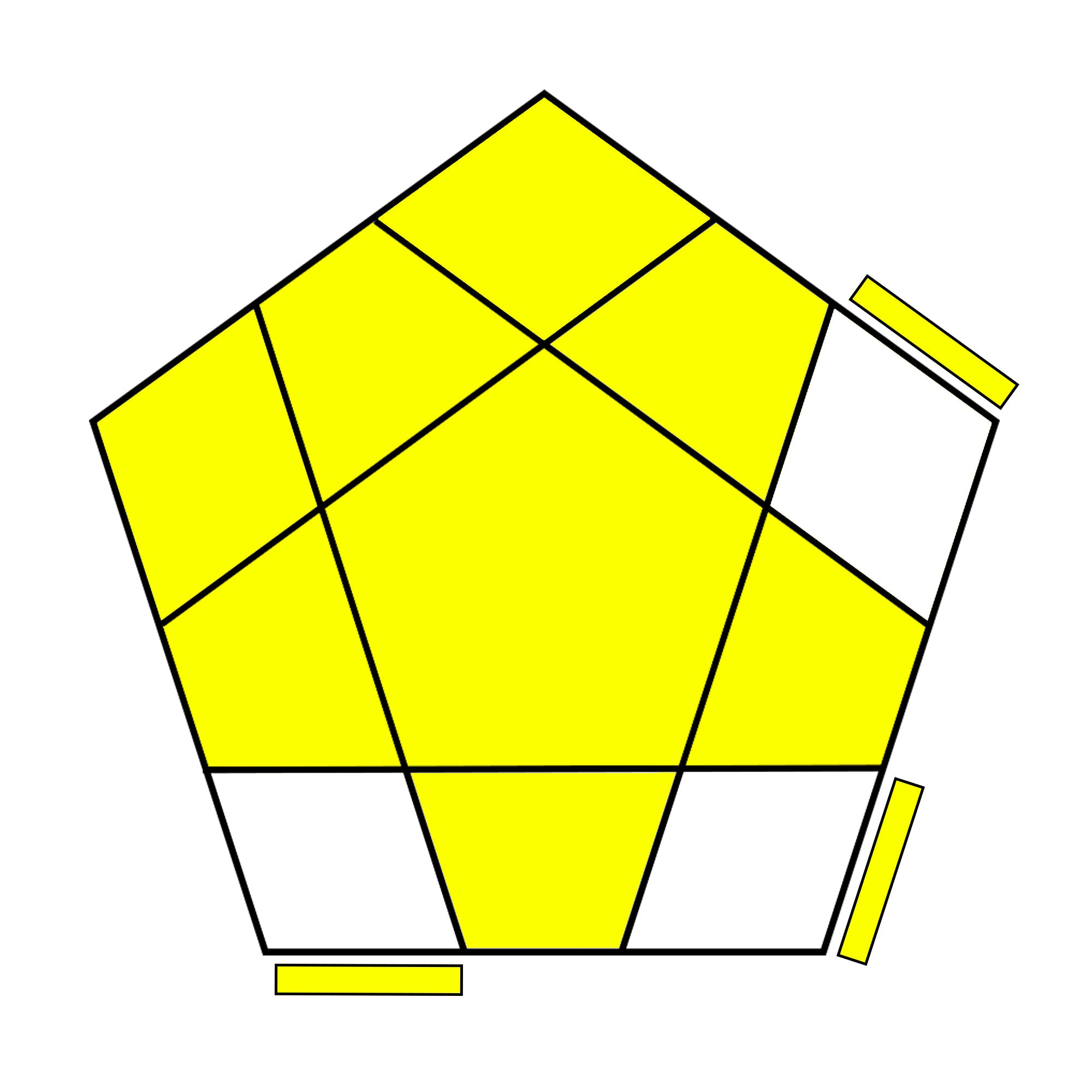R' U' R U' R' U2 R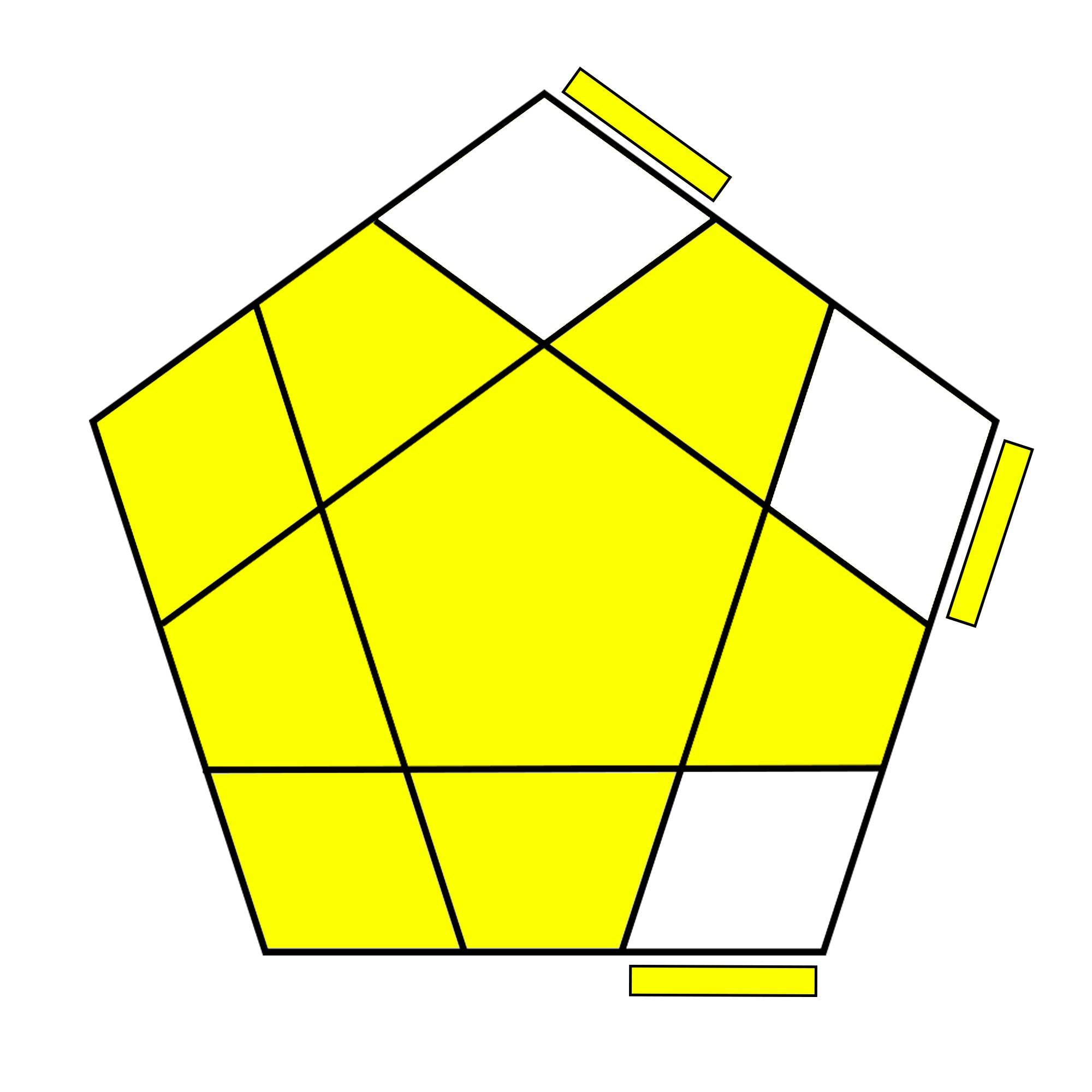R U R' U R U2' R'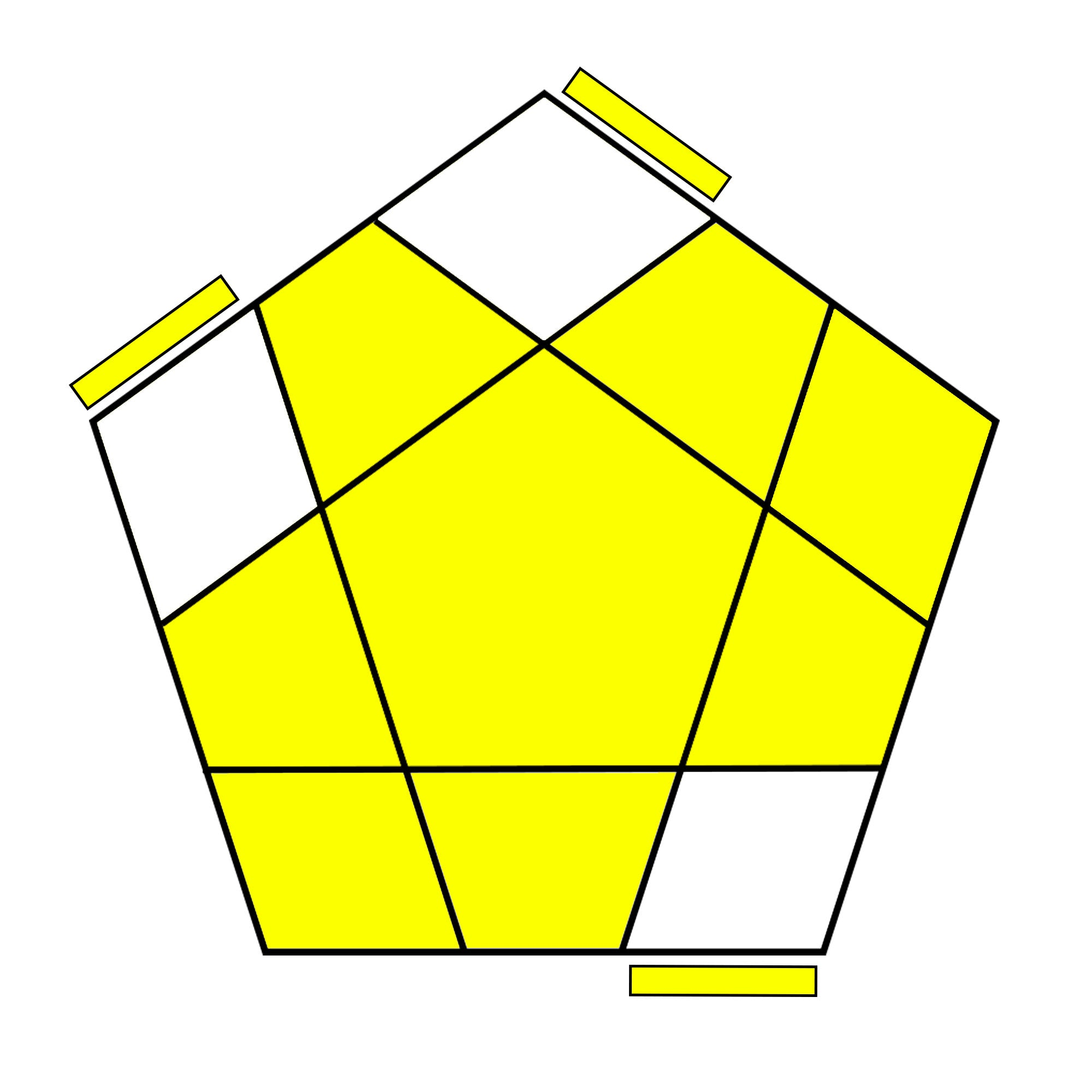R U R' U2 R U2 R'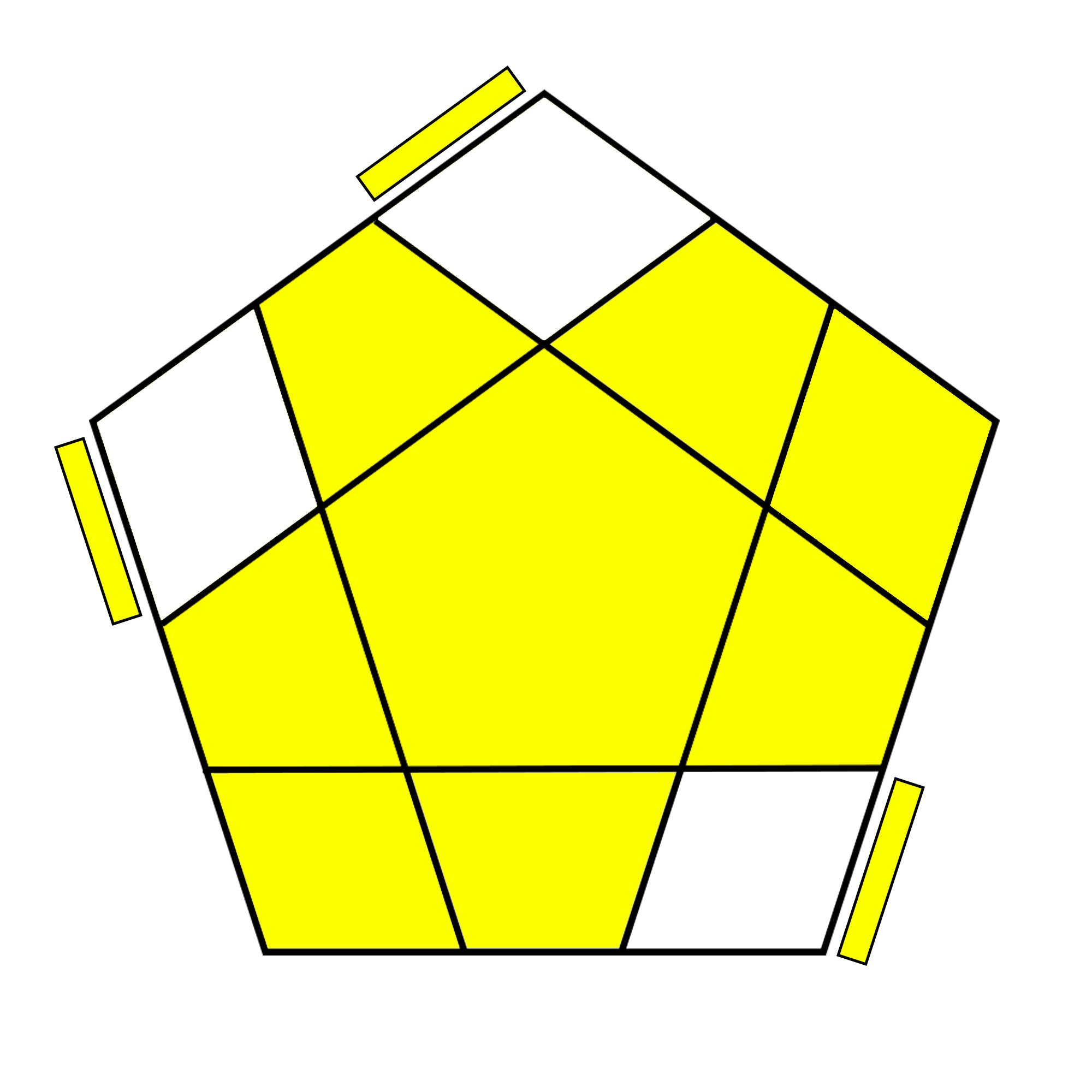R U2 R' U' R U' R'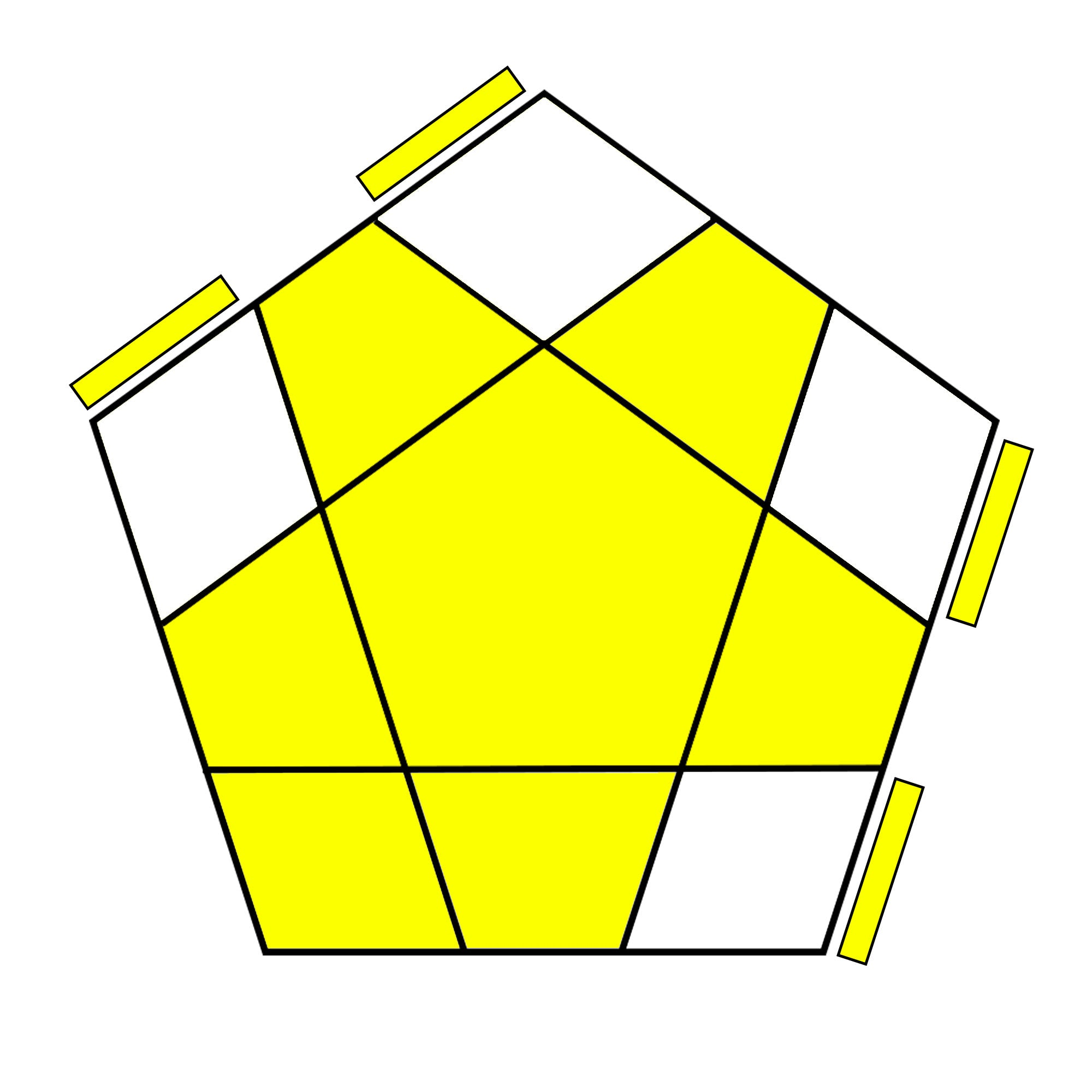( R U R' U) ( R U' R' U) (R U2' R')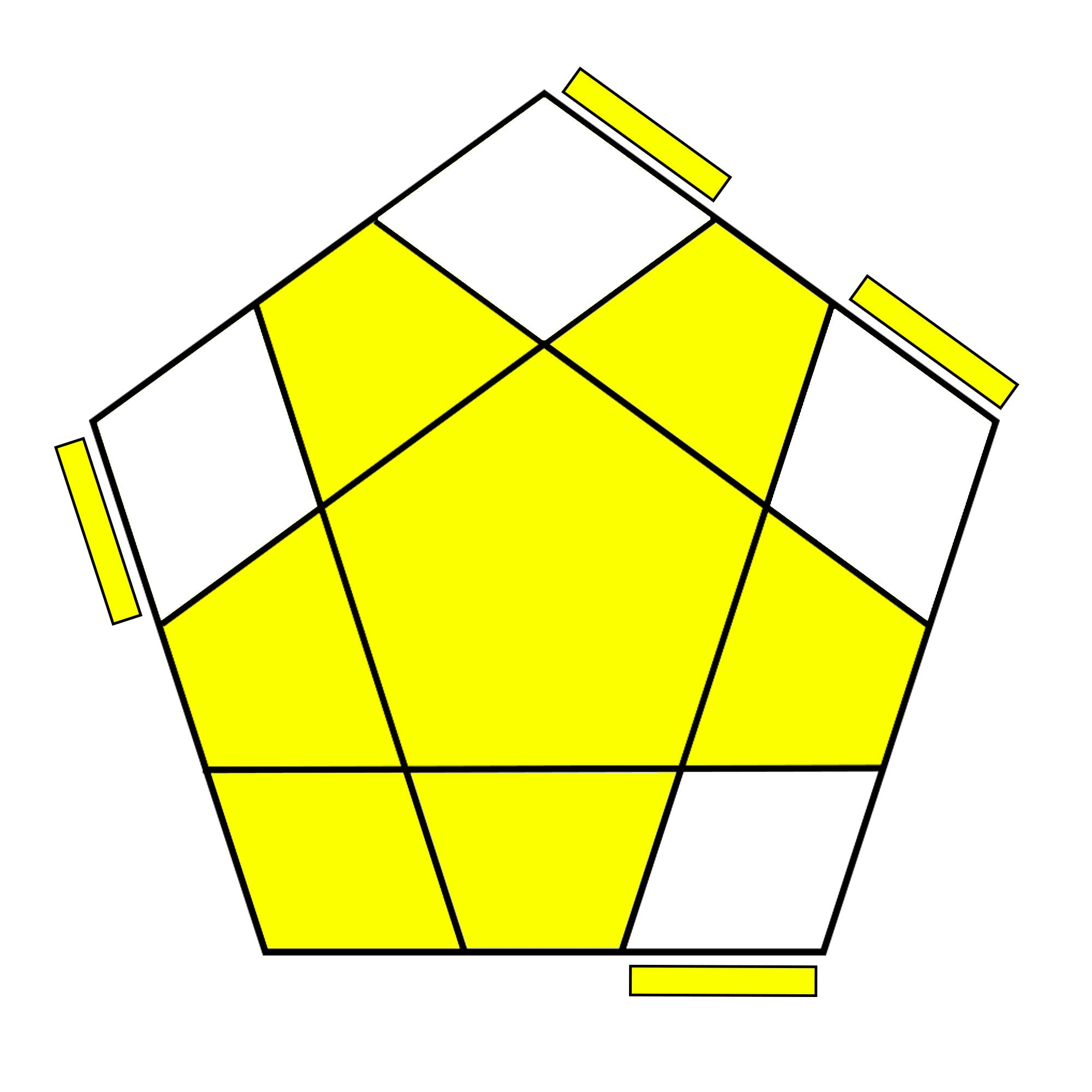( R U2 R' U') ( R U R' U') ( R U' R')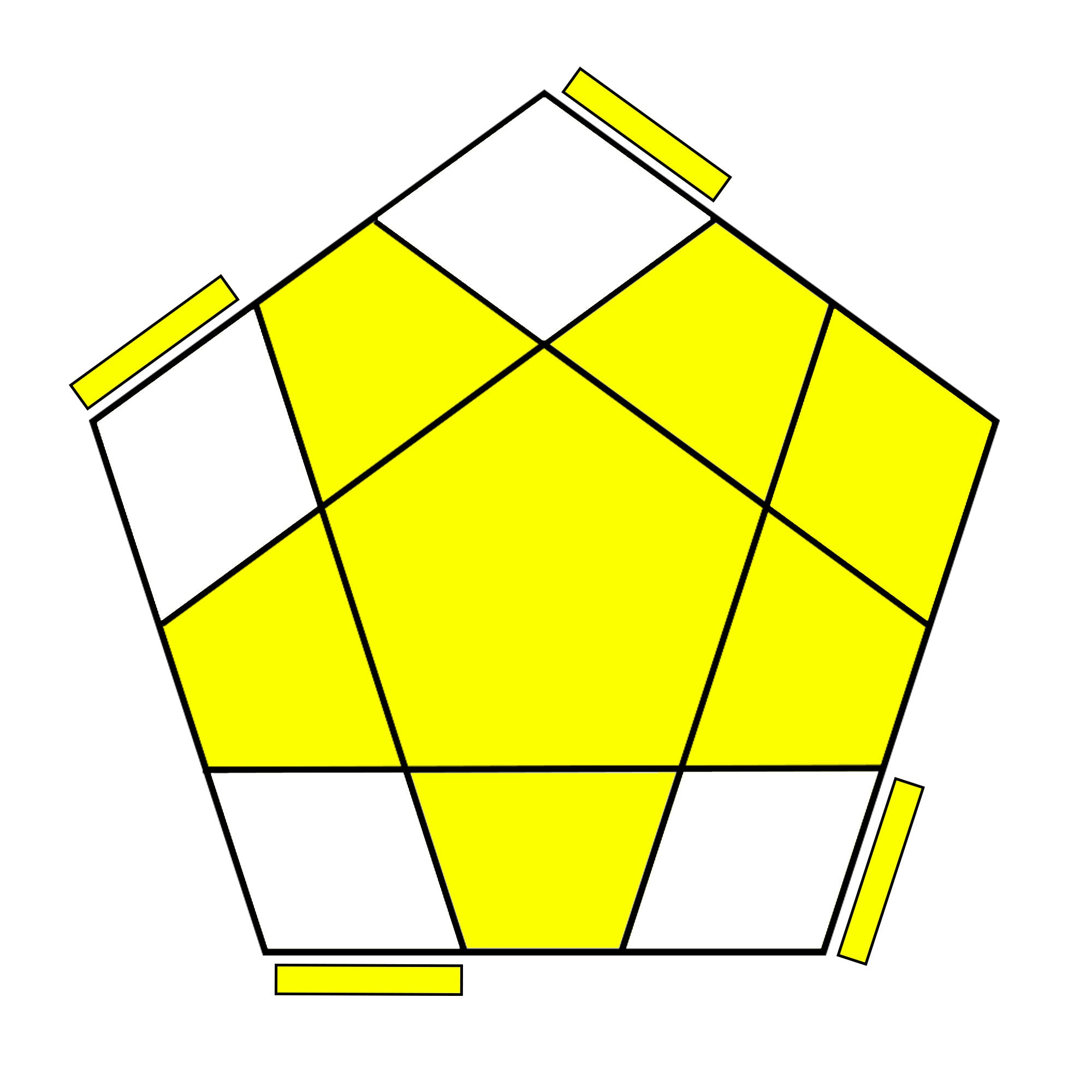(R U2 R' U' R U' R2') ( U' R U' R' U2 R)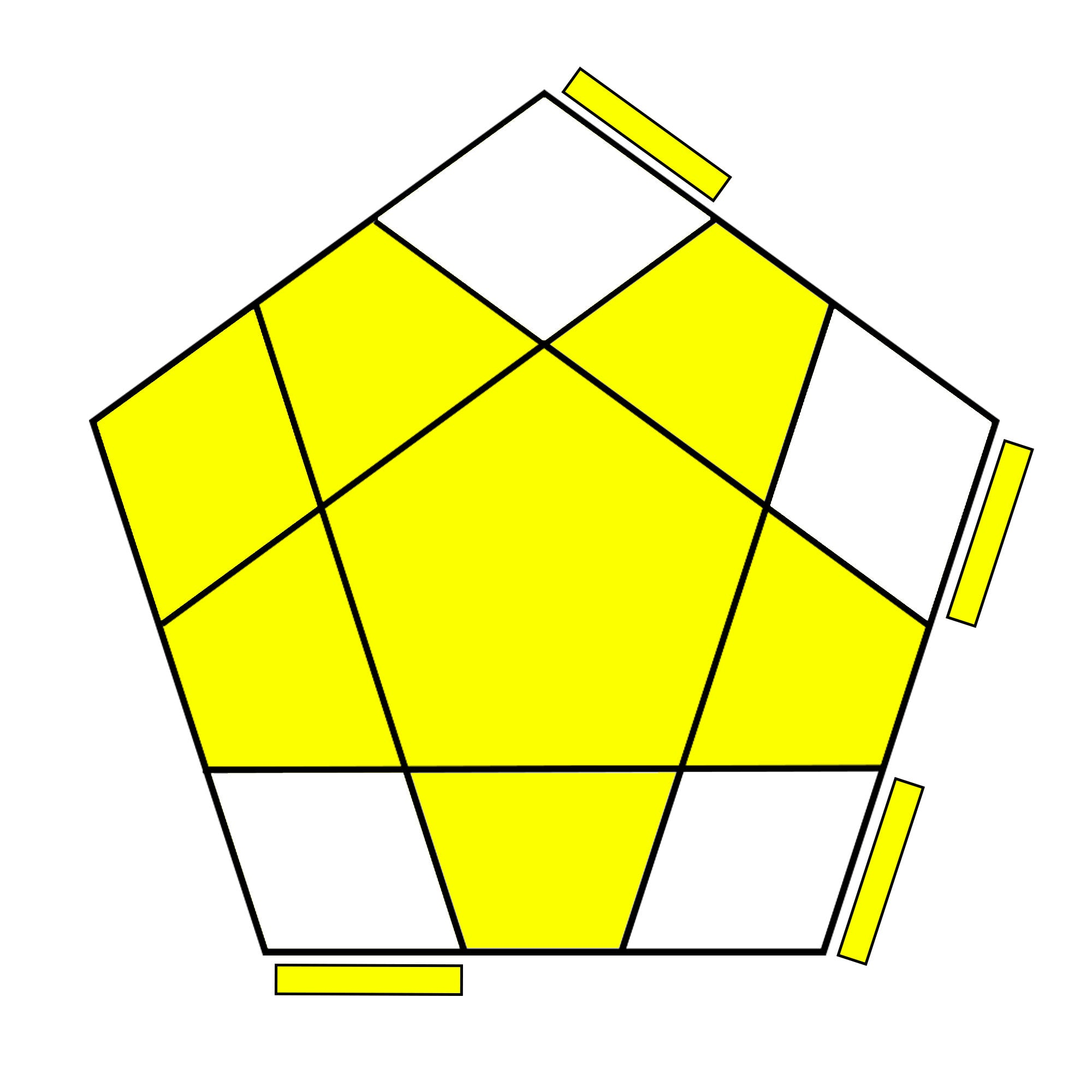( R U R' U) (R U R' U') (R U2' R')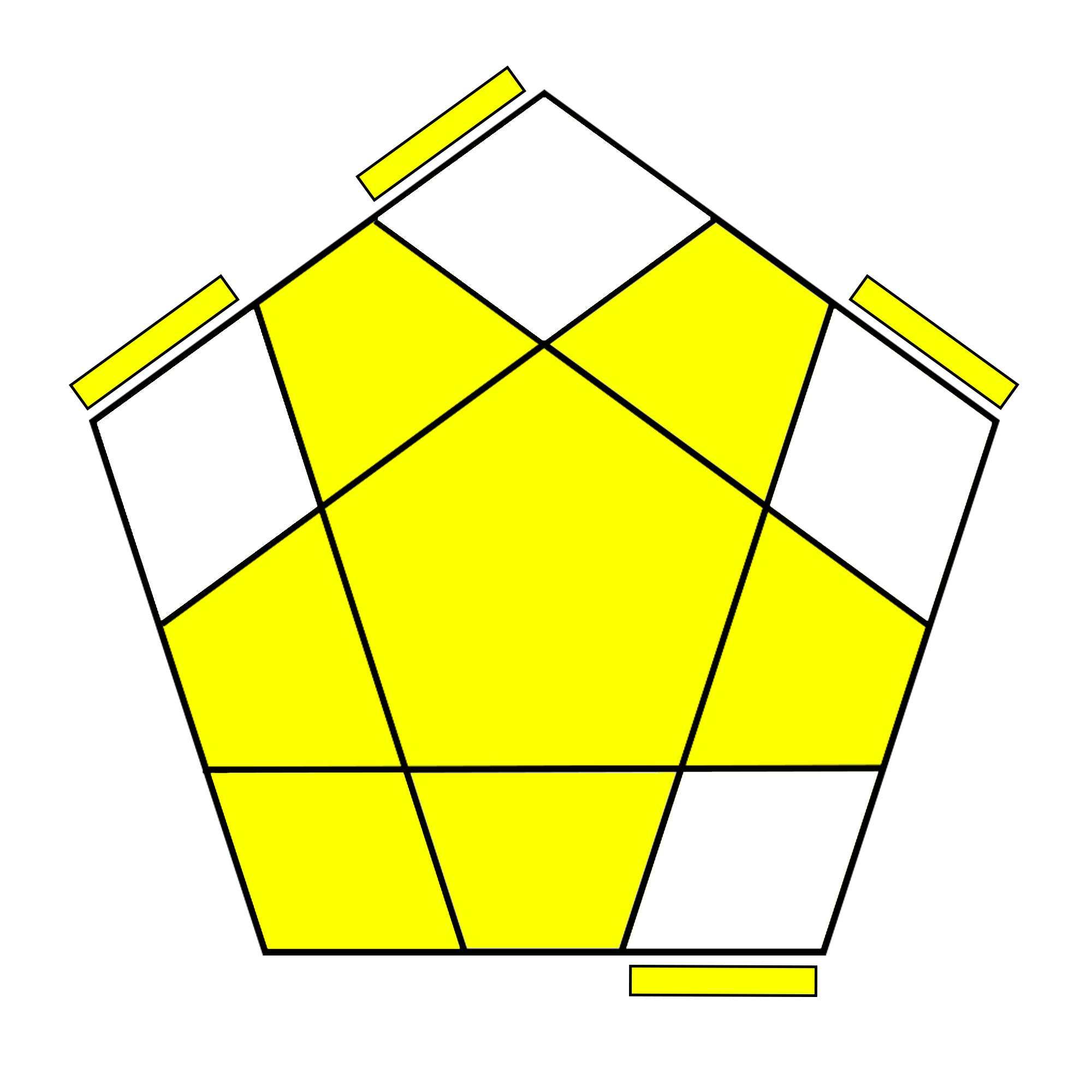R U2 R2' U' R2 U' R2' U2 R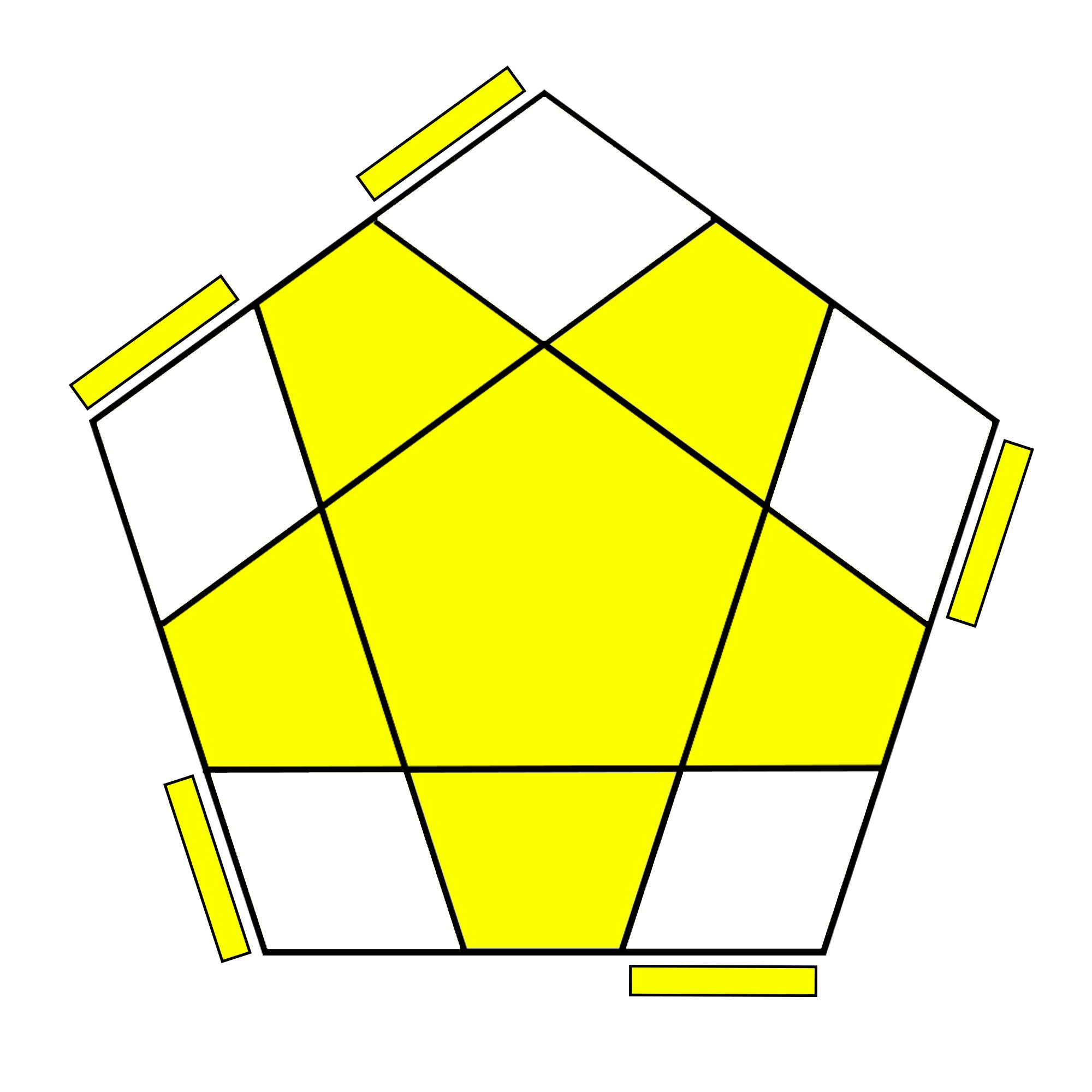( R U R' U2) ( R U2' R') (U R U2' R')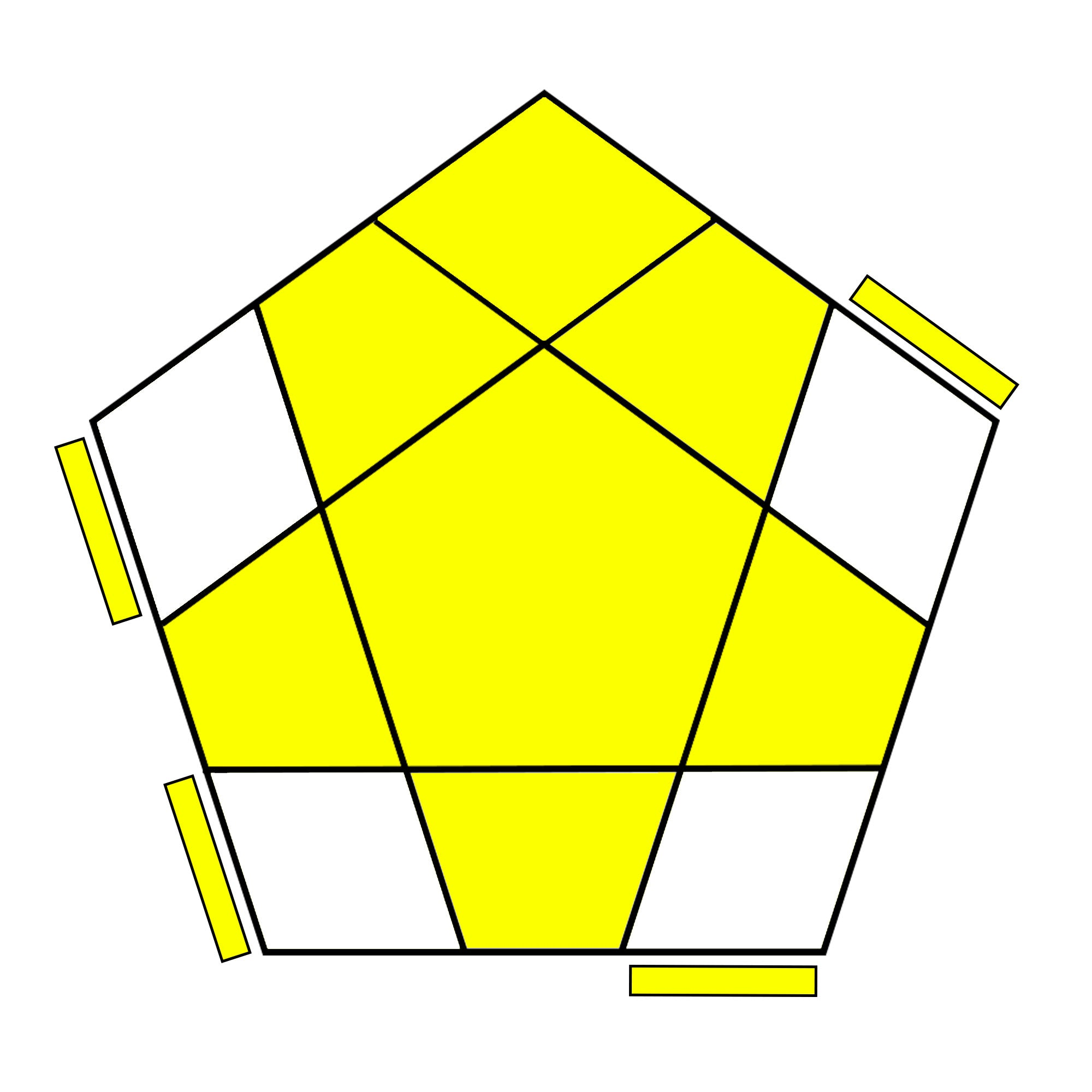R' U2' R2 U R2' U R2 U2' R'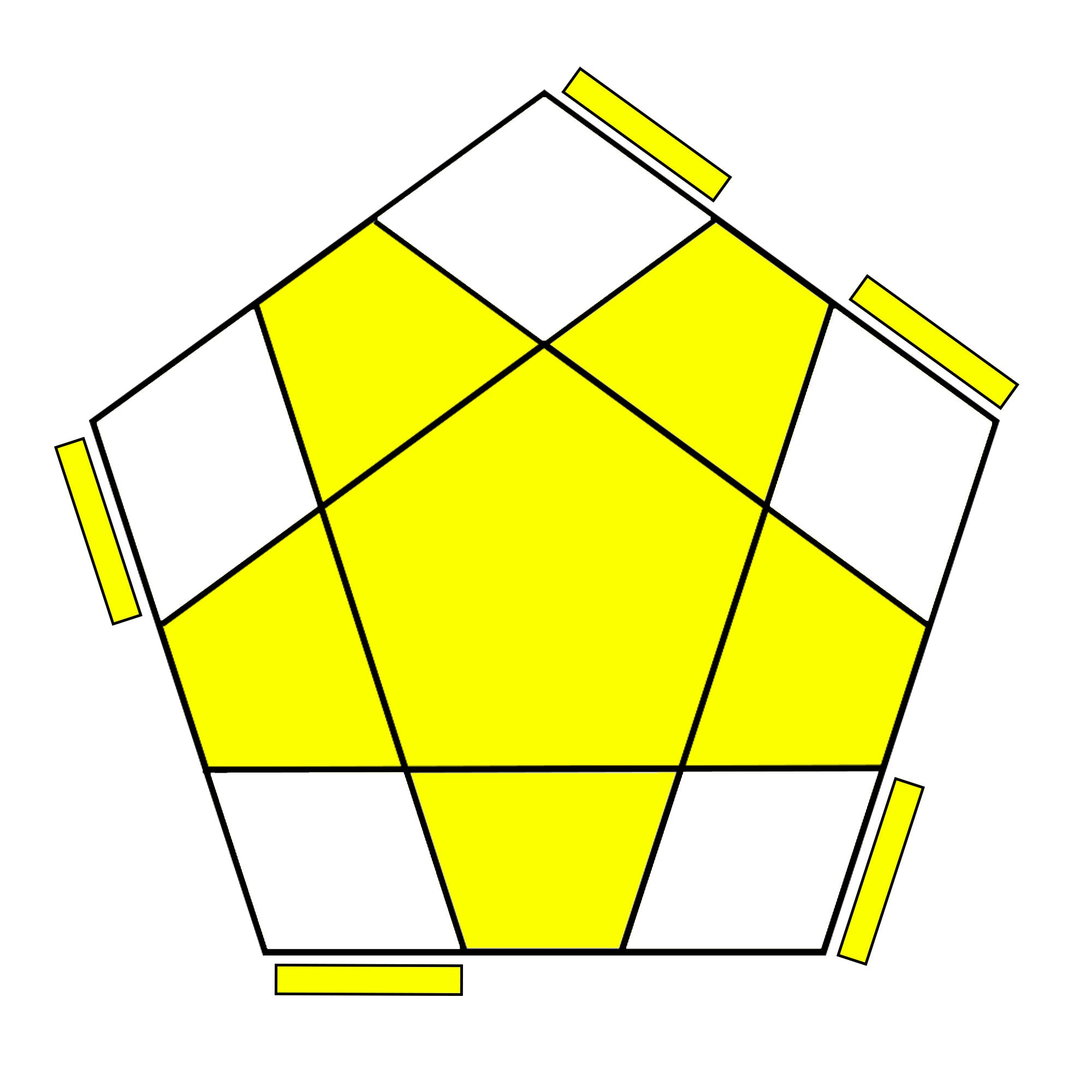( R U2 R' U') ( R U2 R' U2) ( R U' R')

### Megaminx Last Layer - Edge Permutation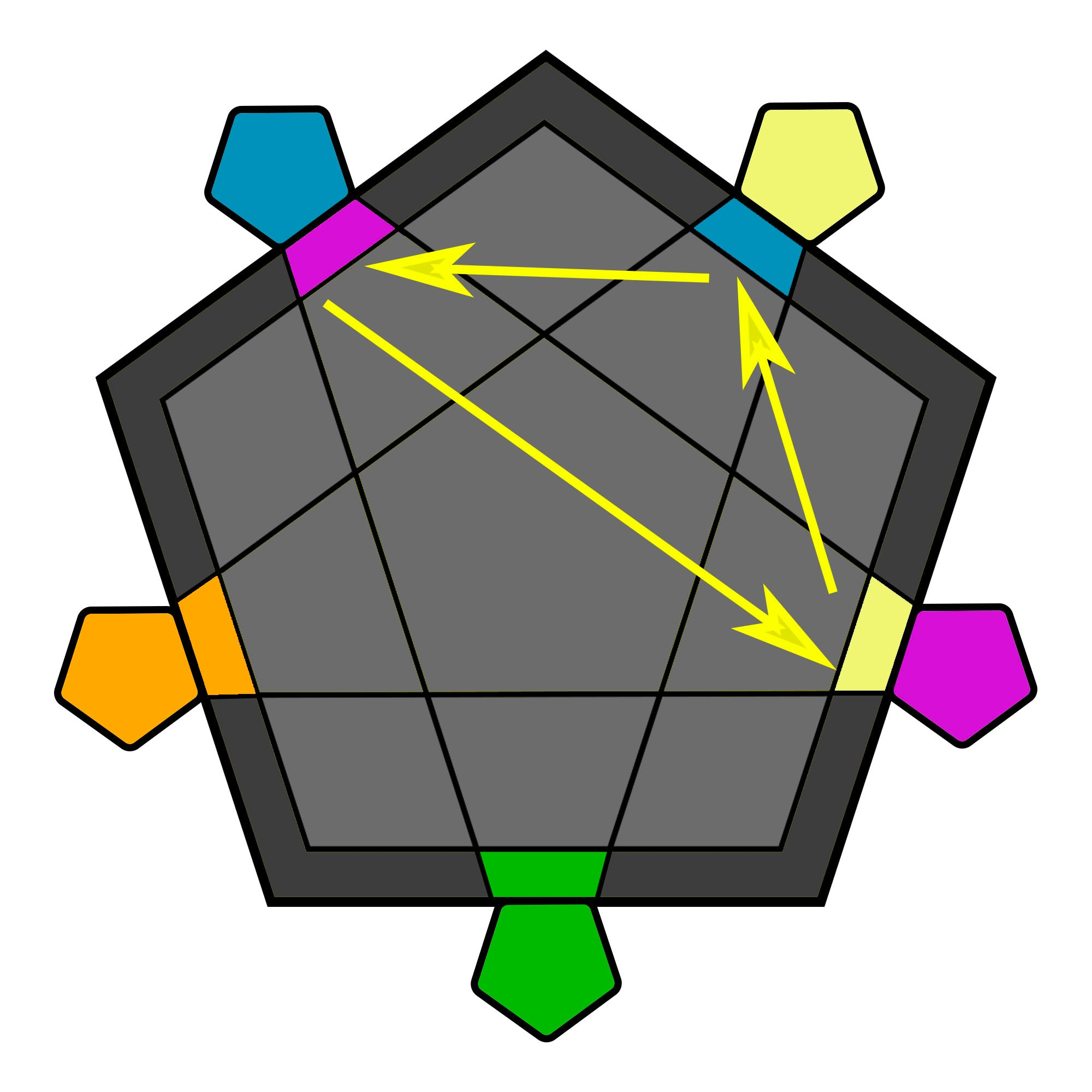(R U R' U) (R' U' R2 U') (R' U R' U) R U2'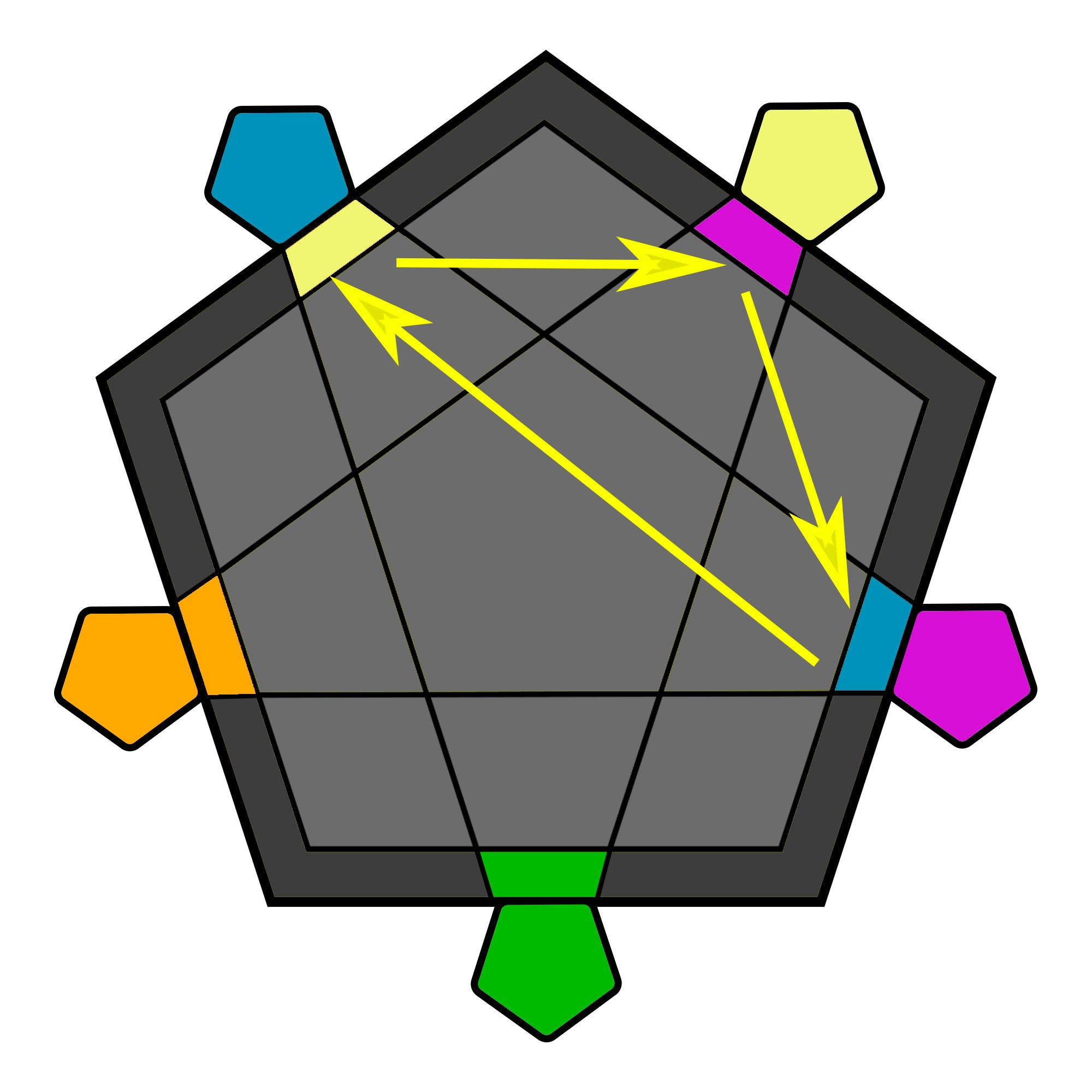(R U R' F') (R U R' U') (R' F R2 U' R')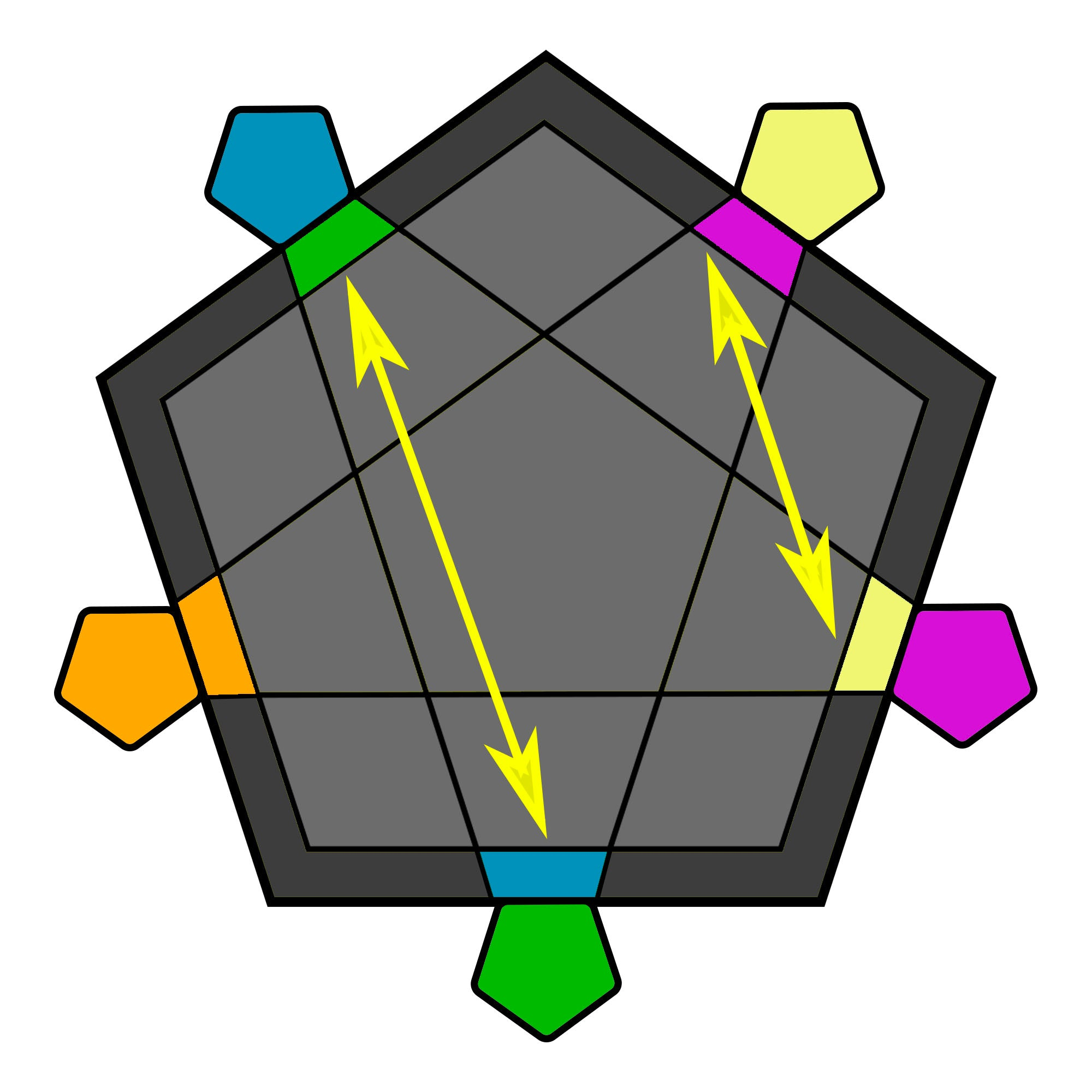(L R U2) (L' U R') (L U' R U2) (L' U2 R')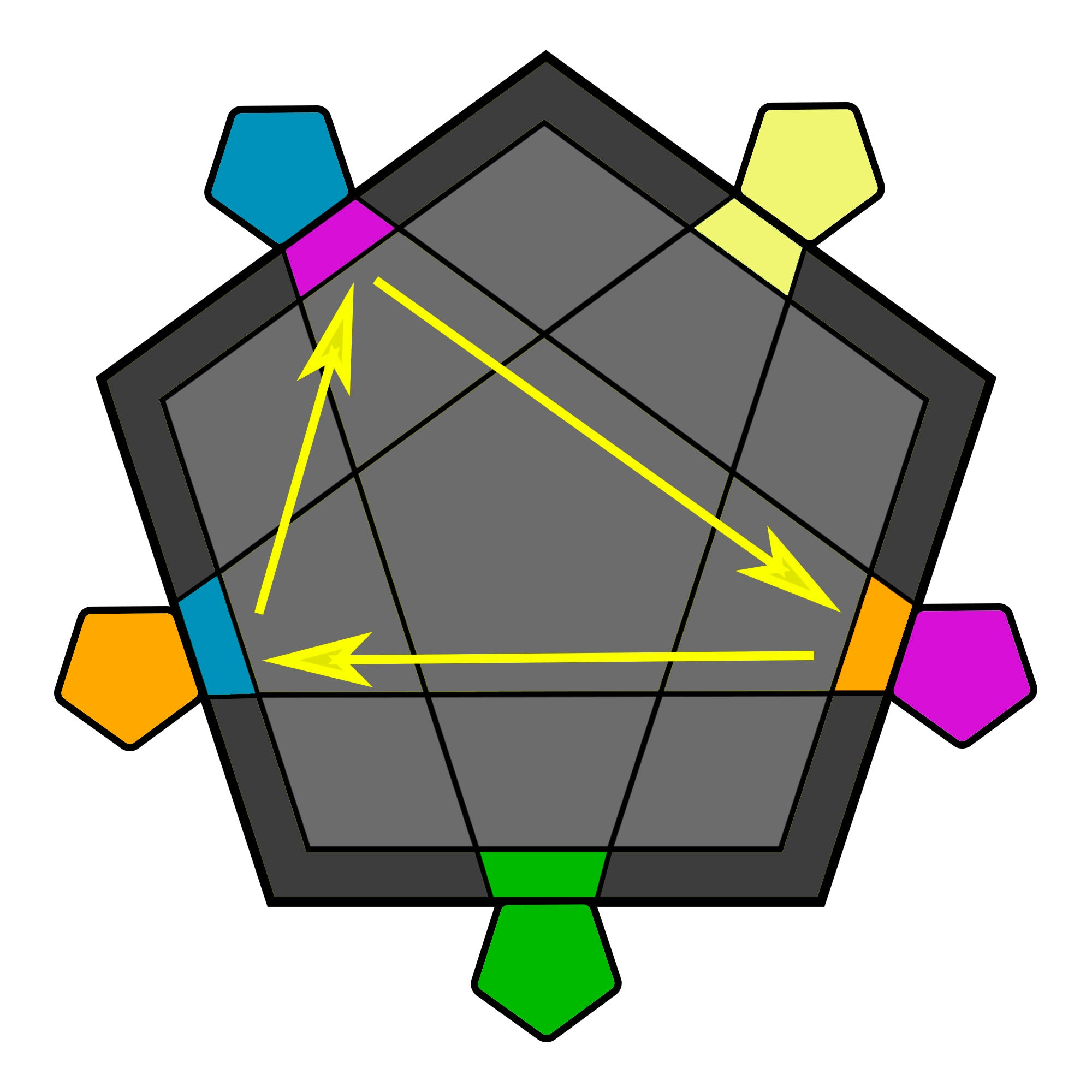R2 U2' R2' U' R2 U2' R2'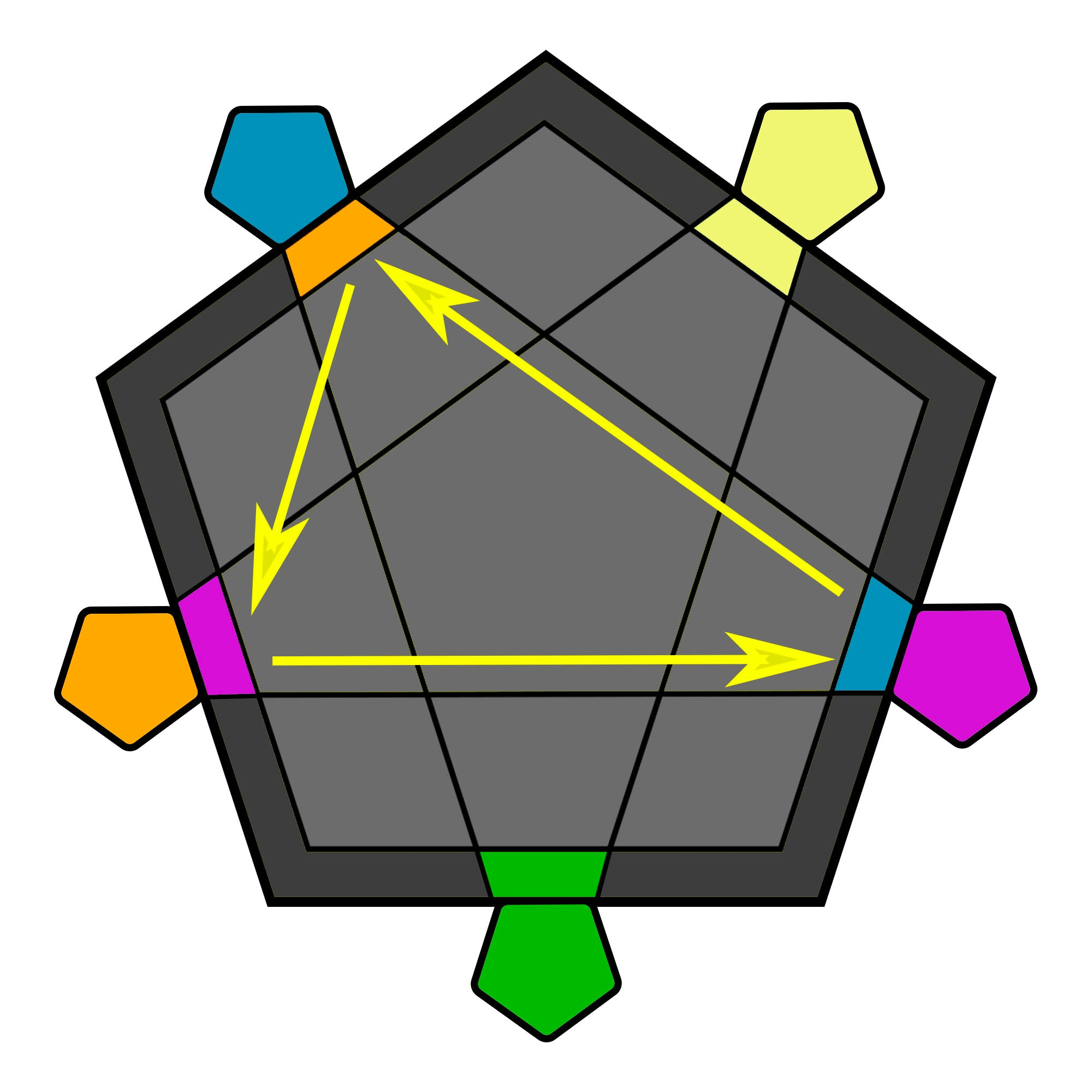R2 U2 R2' U R2 U2 R2'

### Megaminx Last Layer - Corner Permutation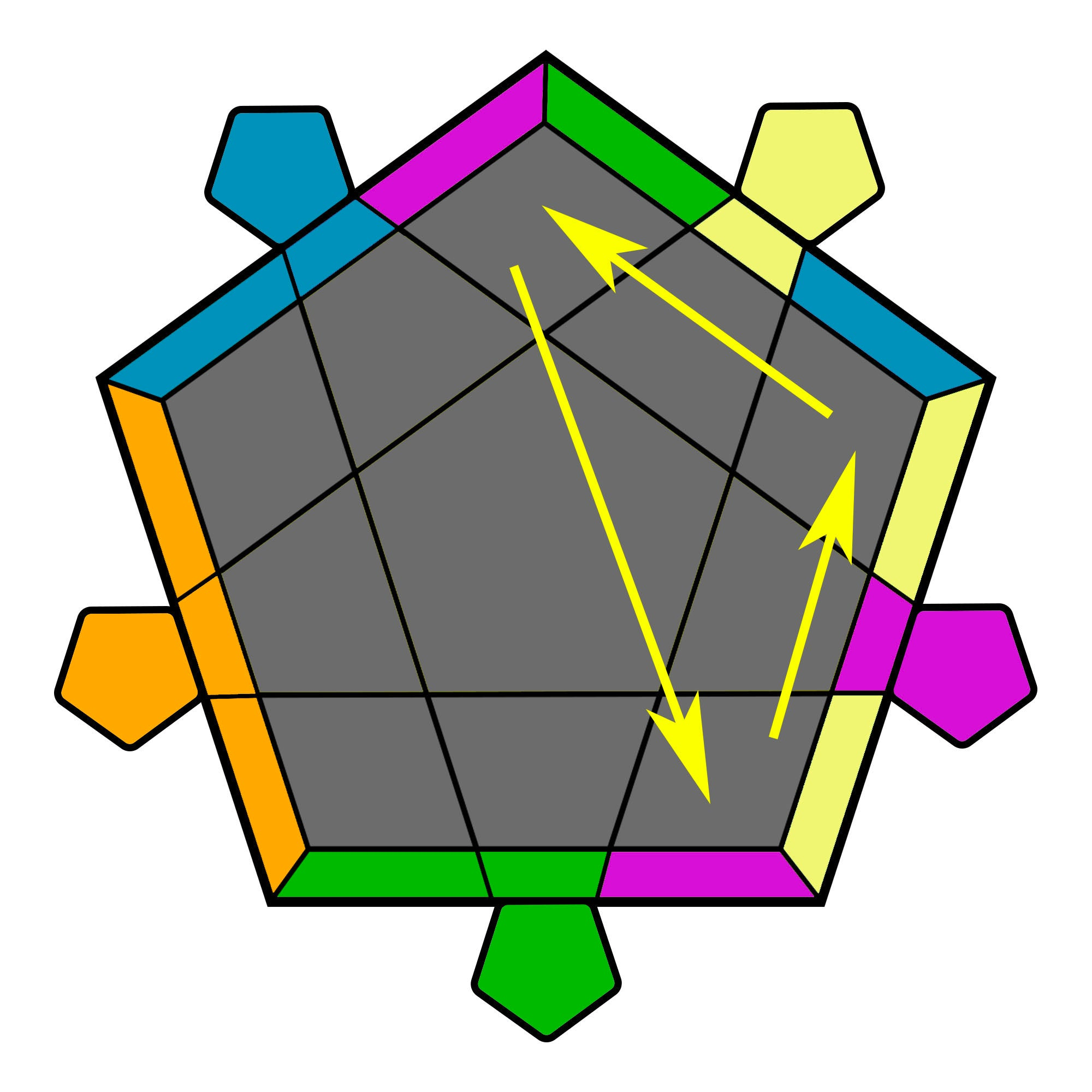(R' F' BR' R) (BR R' F R) (BR' R' BR R)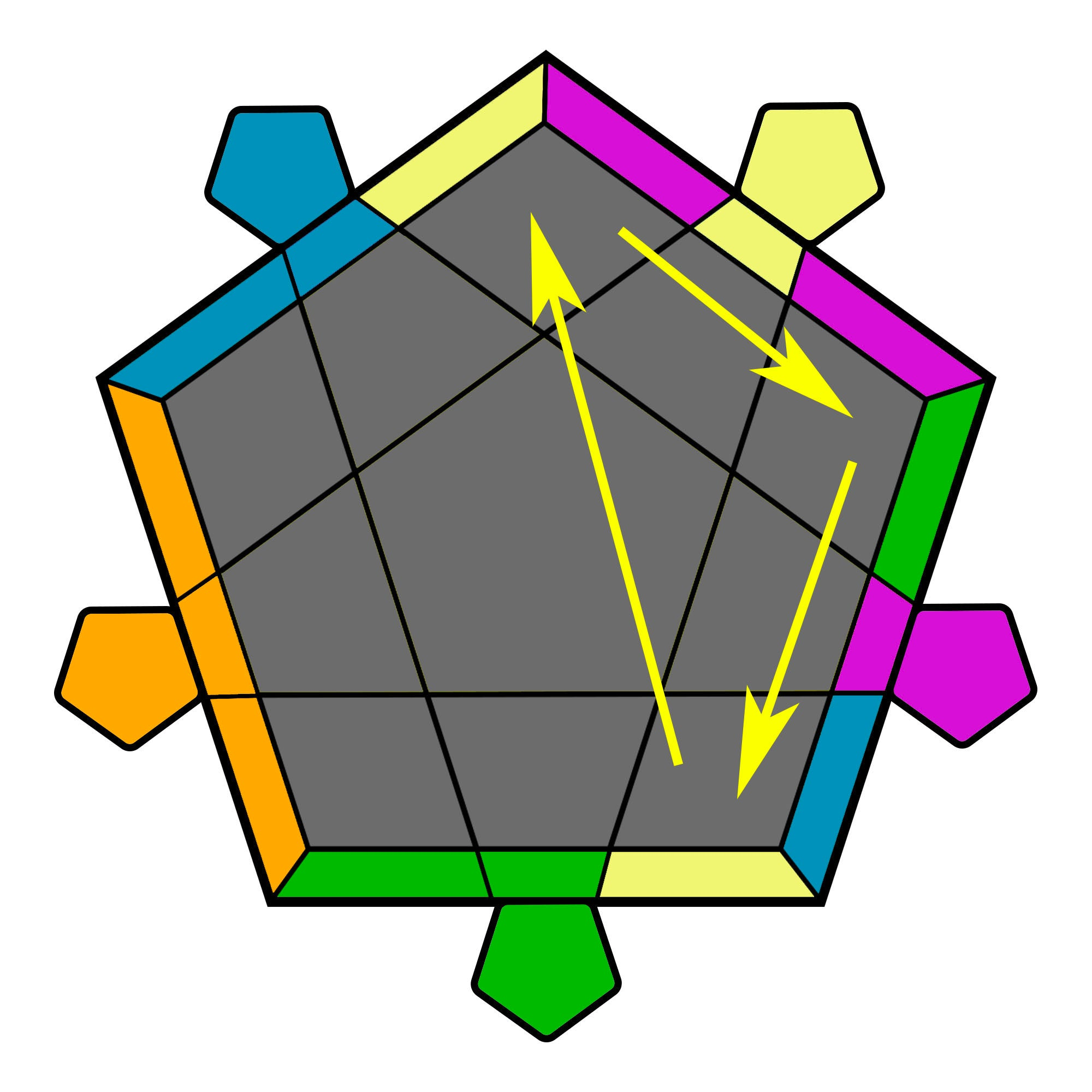(R' BR' R BR) (R' F' R BR')(R' BR F R)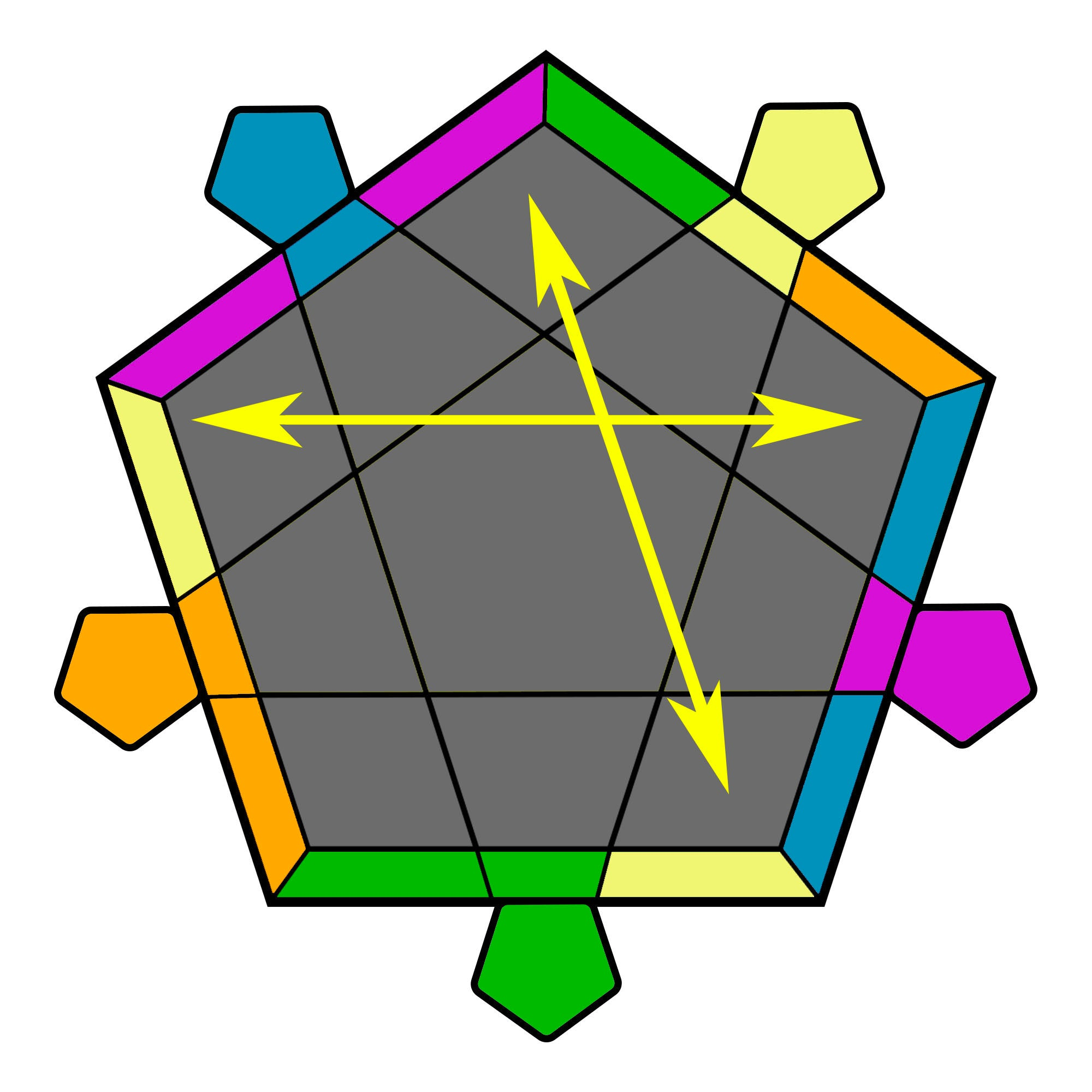y L' (R U2 R' U') (R U R' U') (R U R' U') RU' R' L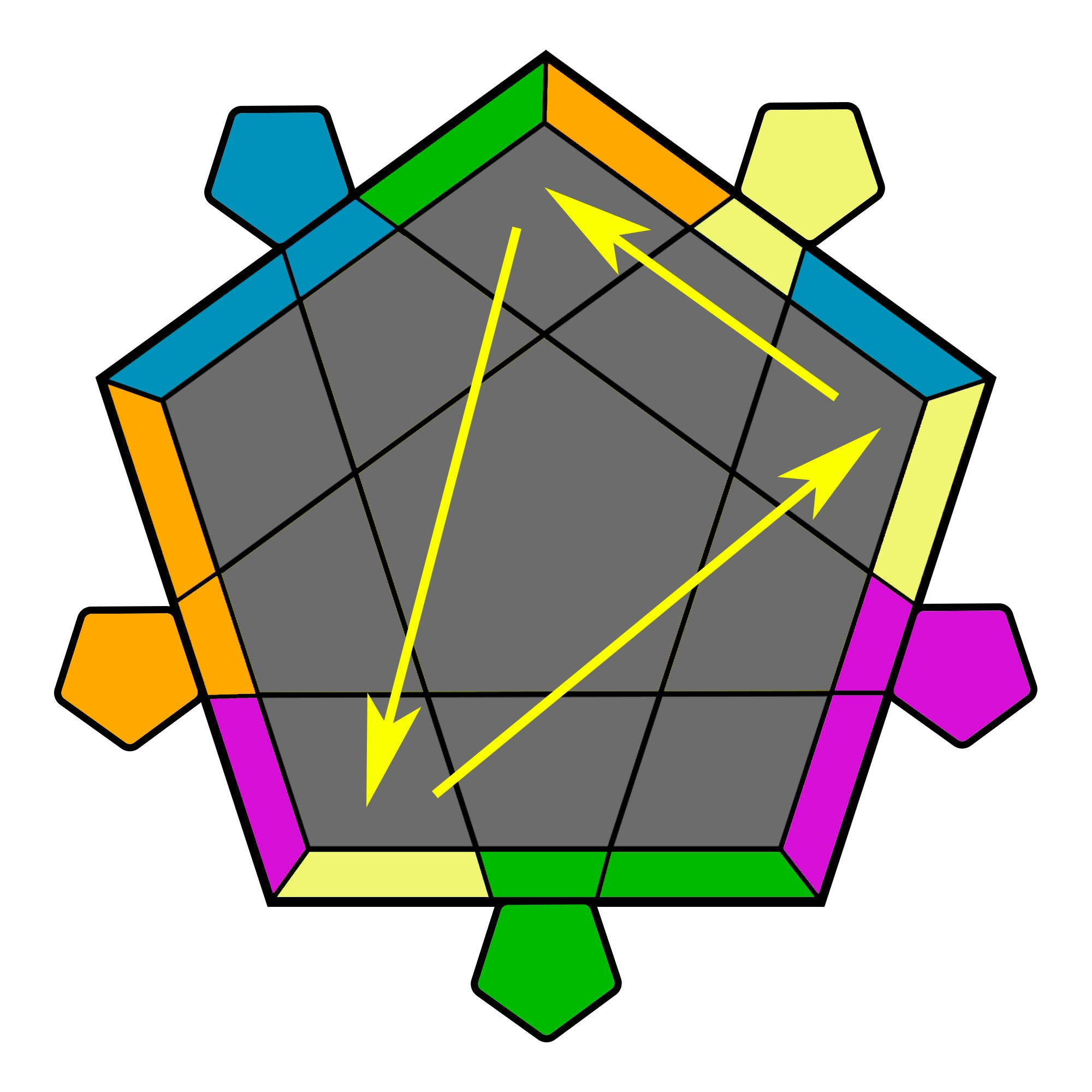BR' R2' (U L U' R) (U L' U' R)BR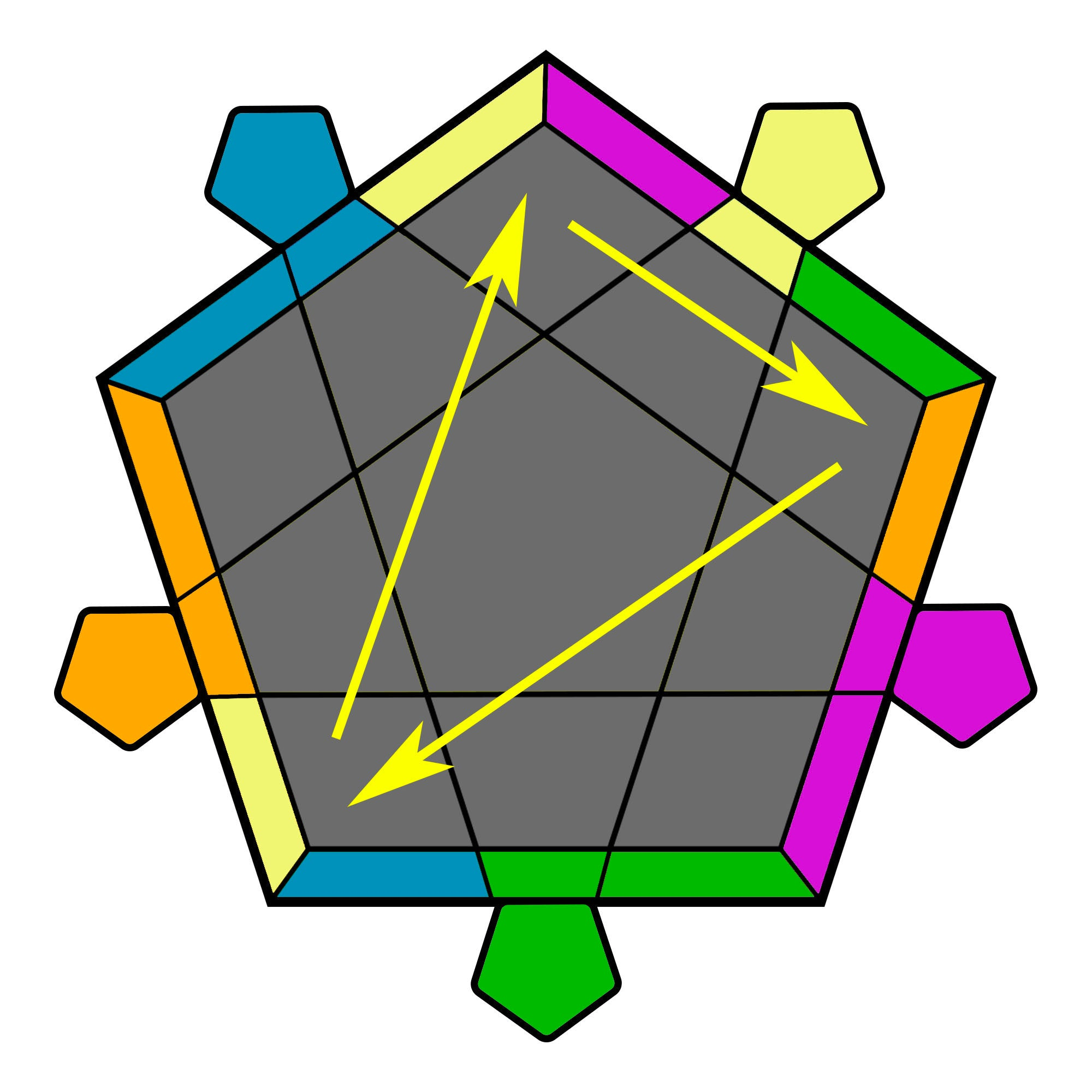BR' (R' U L U') (R' U L' U')R2 BR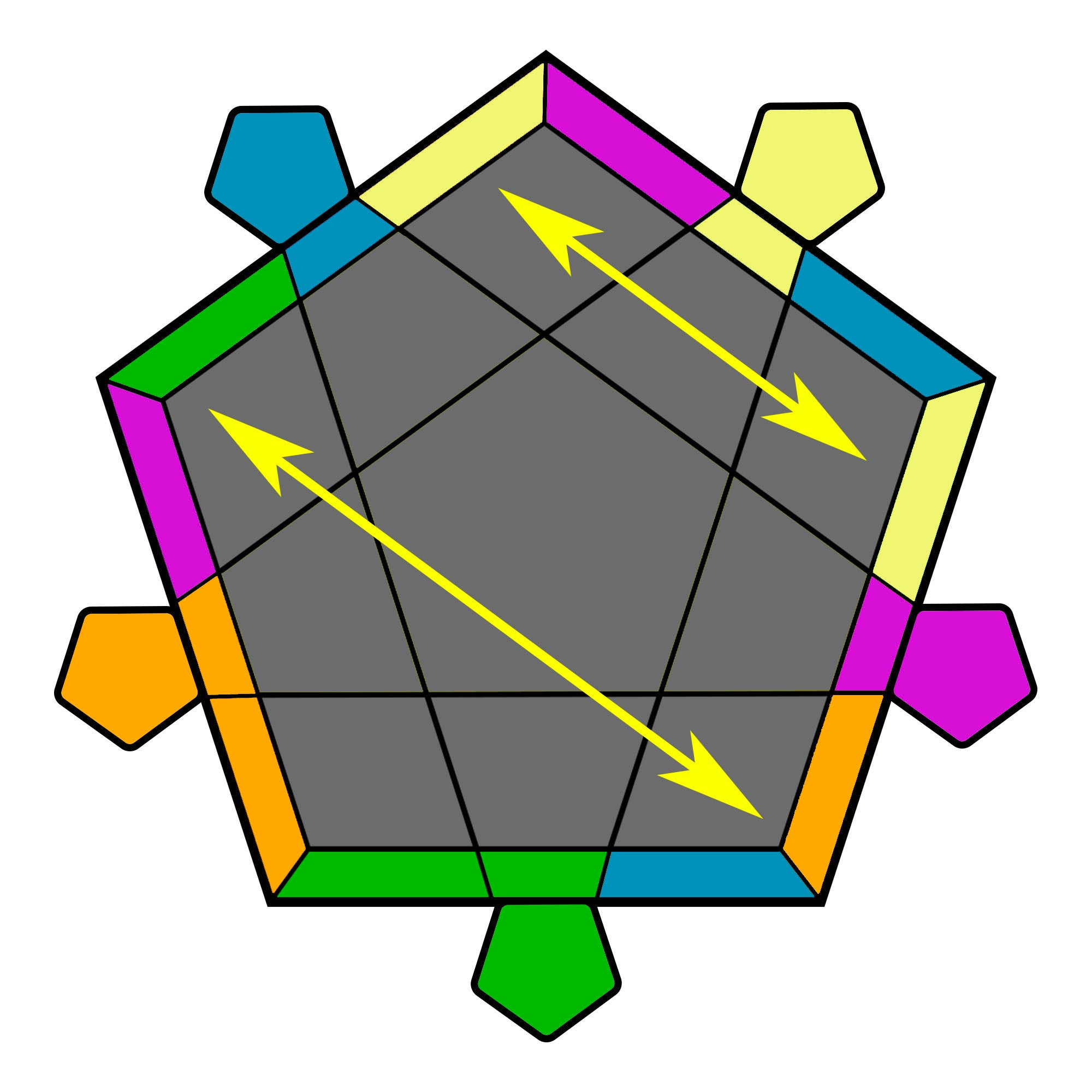R2 U R' U' y (R U R' U') (R U R' U') (R UR') y' R U' R2'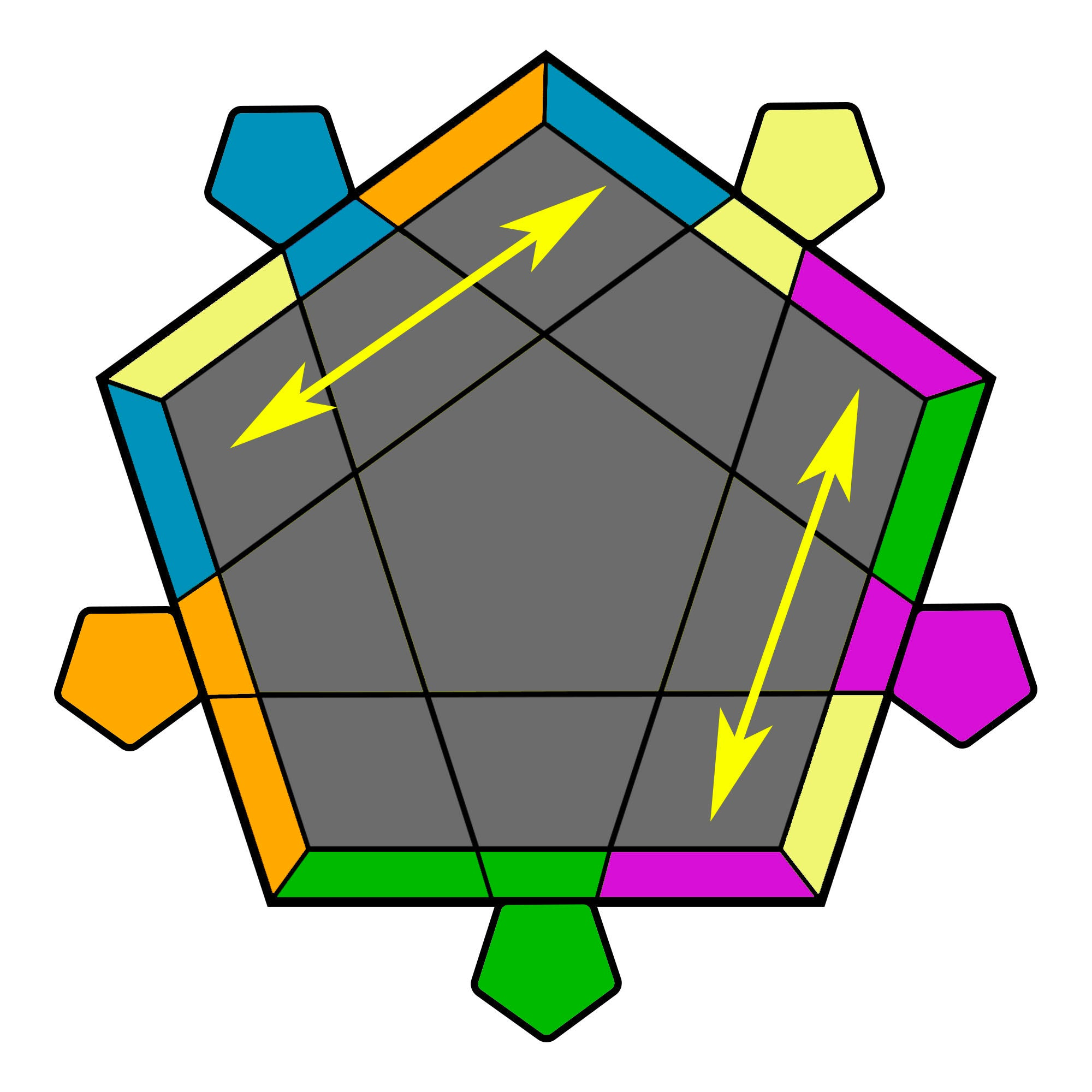(R U R' U) (R' U' R F') (R U R' U') (R' FR2 U') (R2' U R U')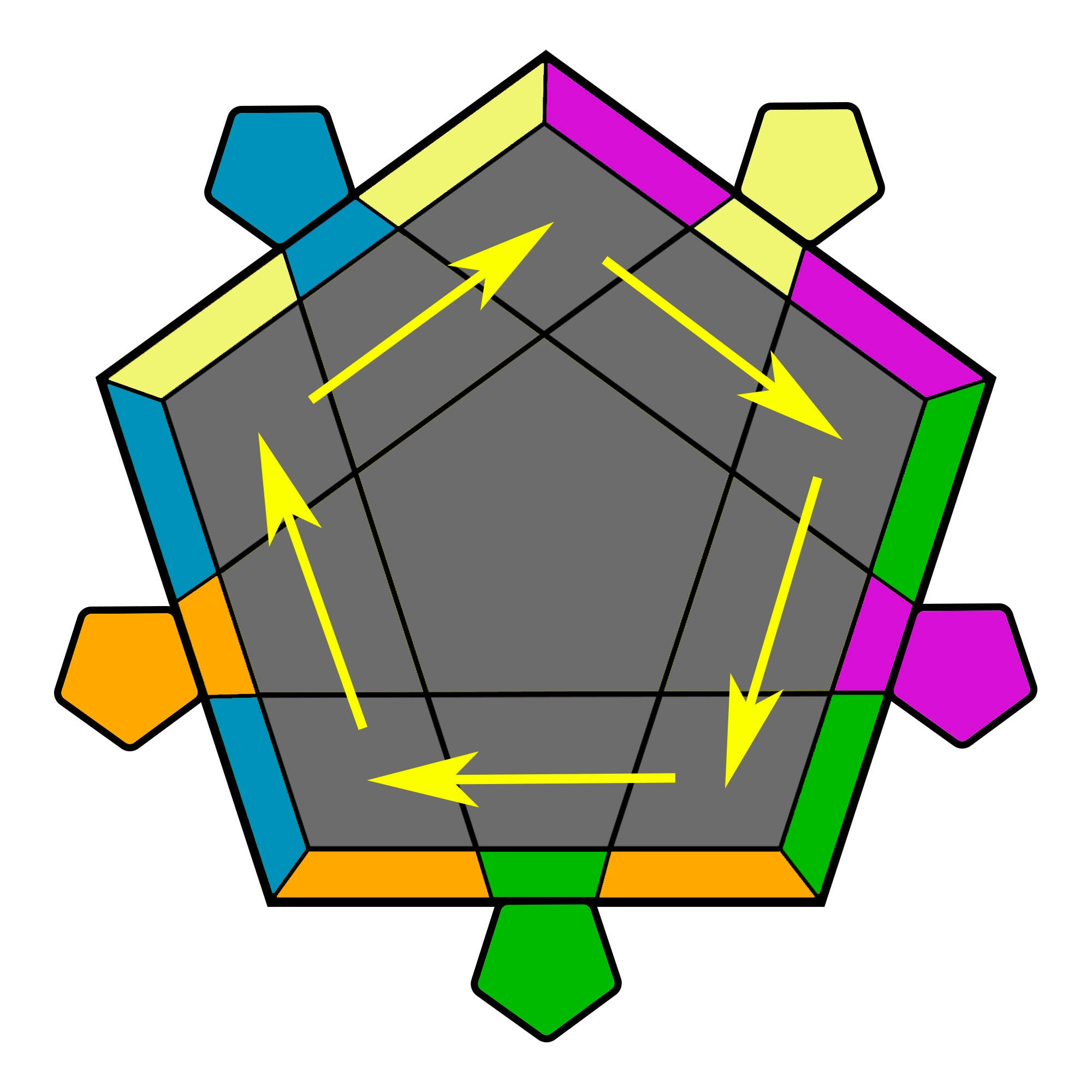'[(R U R' U) (R' U' R2 U') (R' UR' U R) U] *2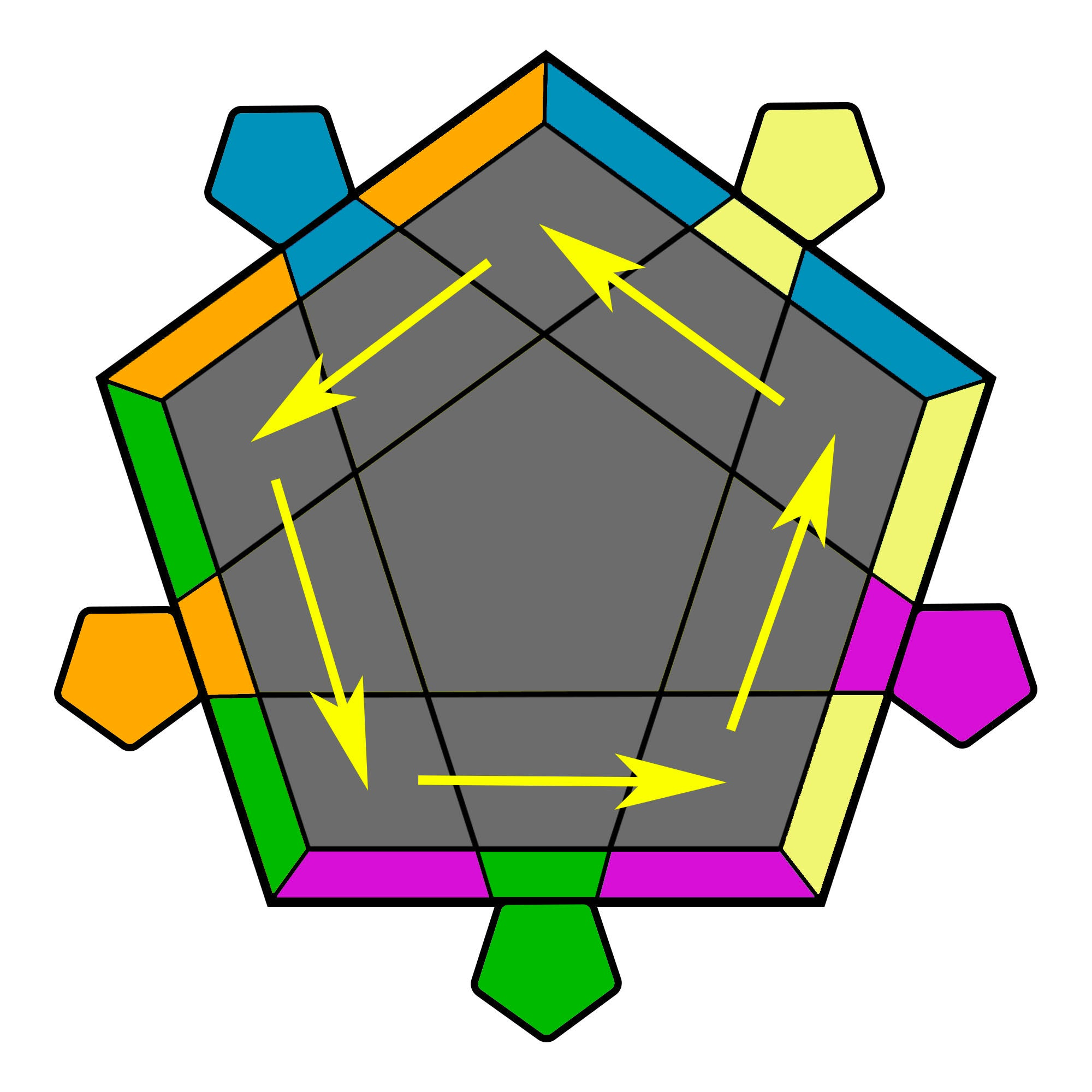F (R U2 R' U' R U' R') F' R'y' (R' U' R U' R' U2 R BR) U'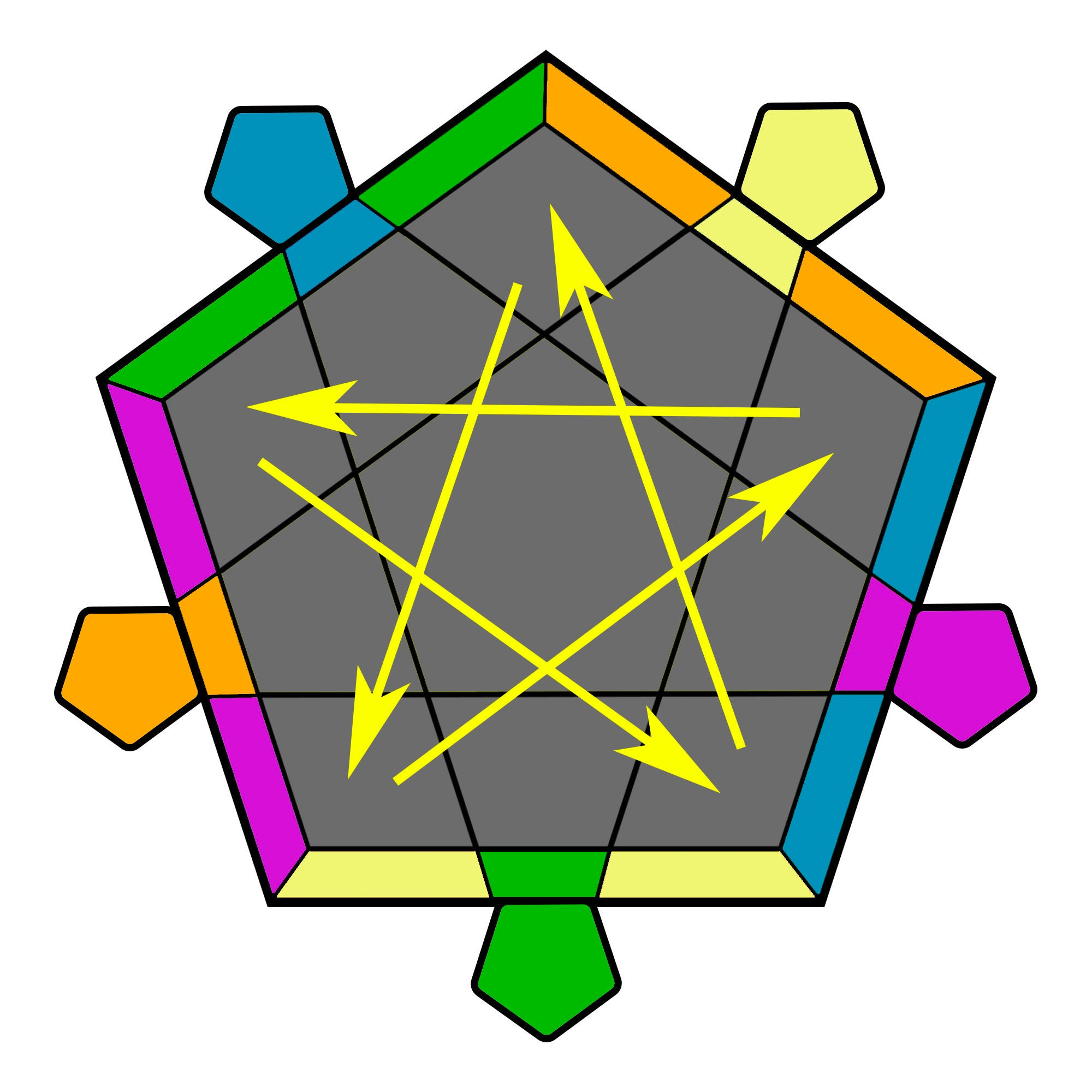R2 U2 R2' U' R2 U' R2' y'R2' U' R2 U' R2' U2 R2 U'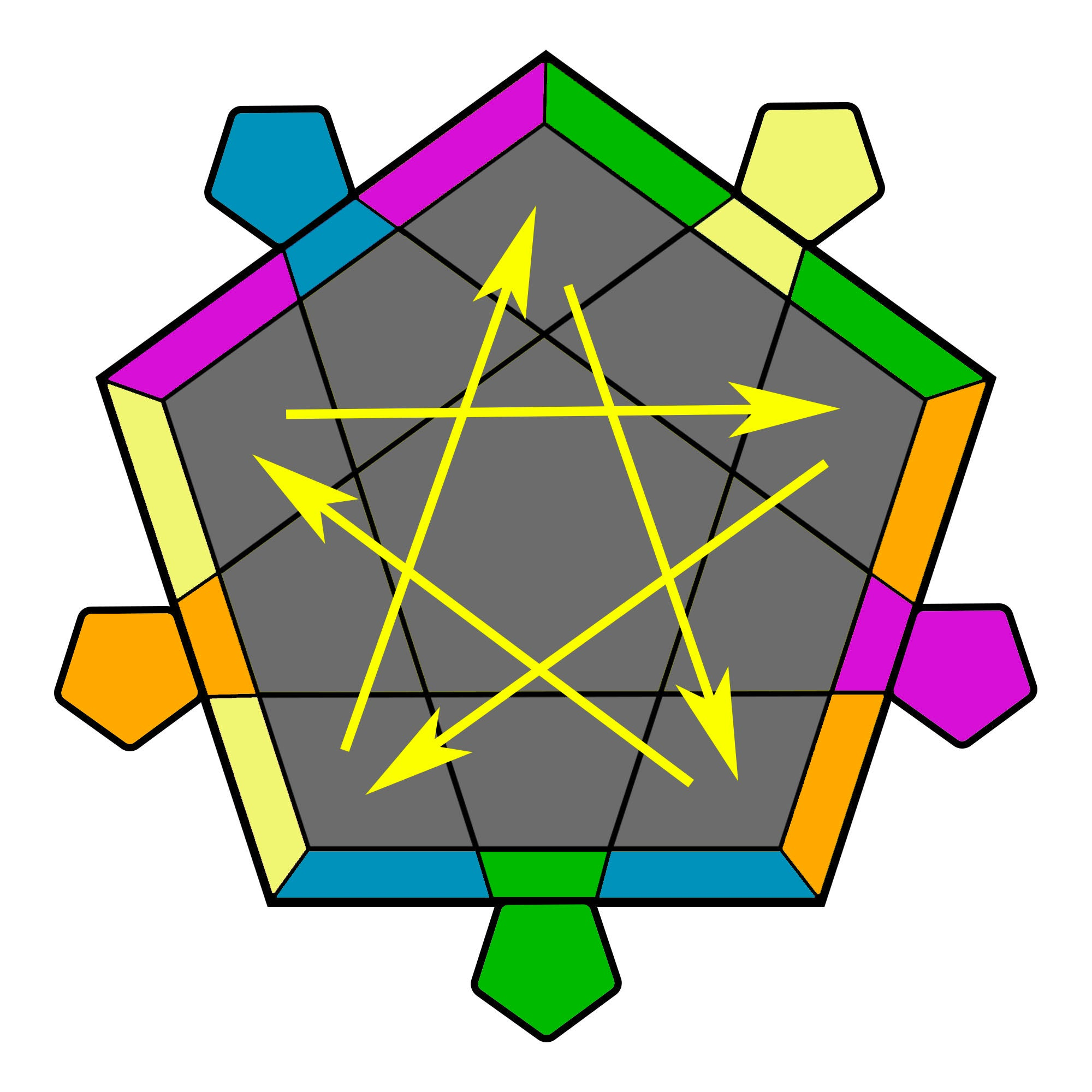R2' U2' R2 U R2' U R2 yR2 U R2' U R2 U2' R2' U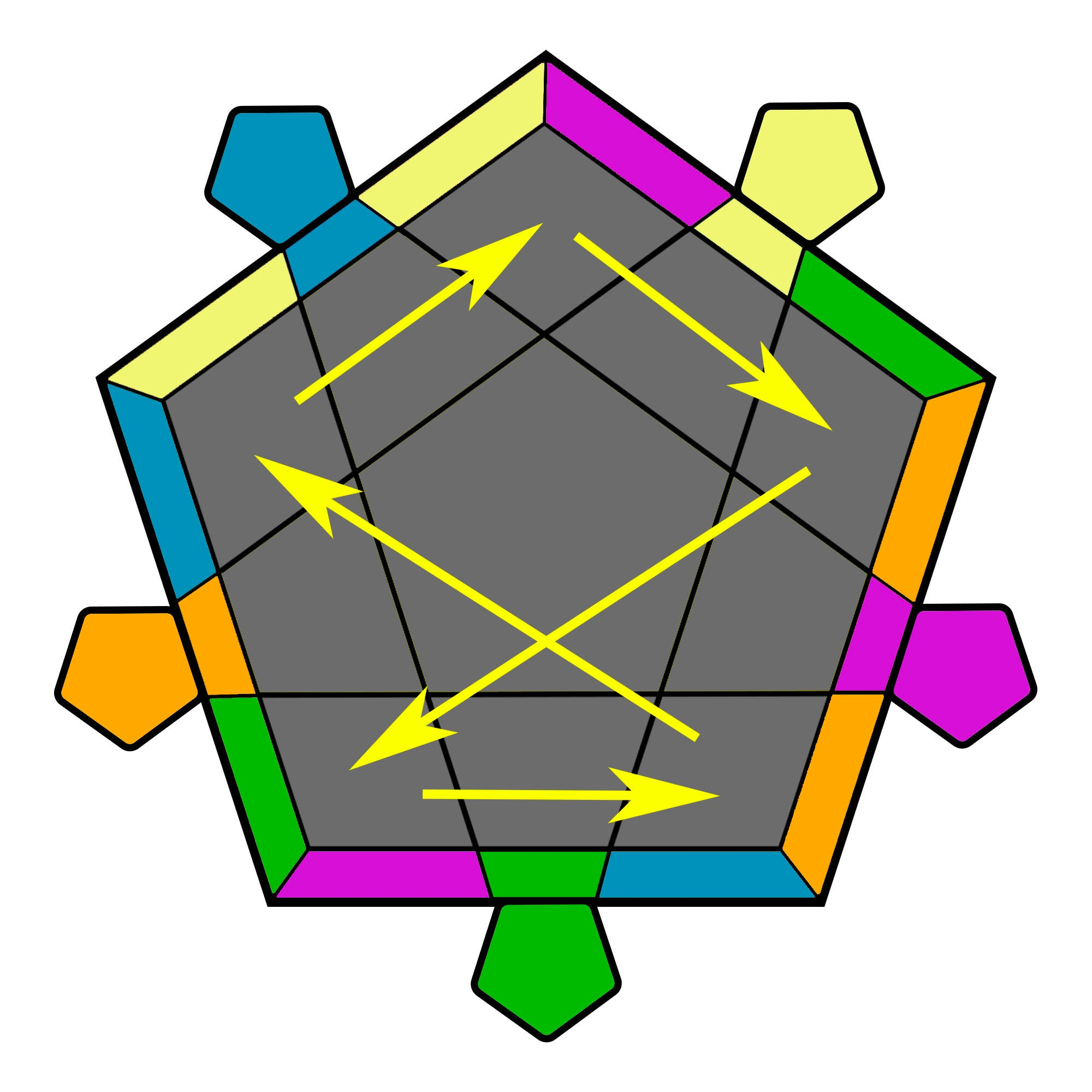R2 U2' R2' U' R2 U2'(R' U R' U') (R' F R2 U')(R' U' R U) R' F'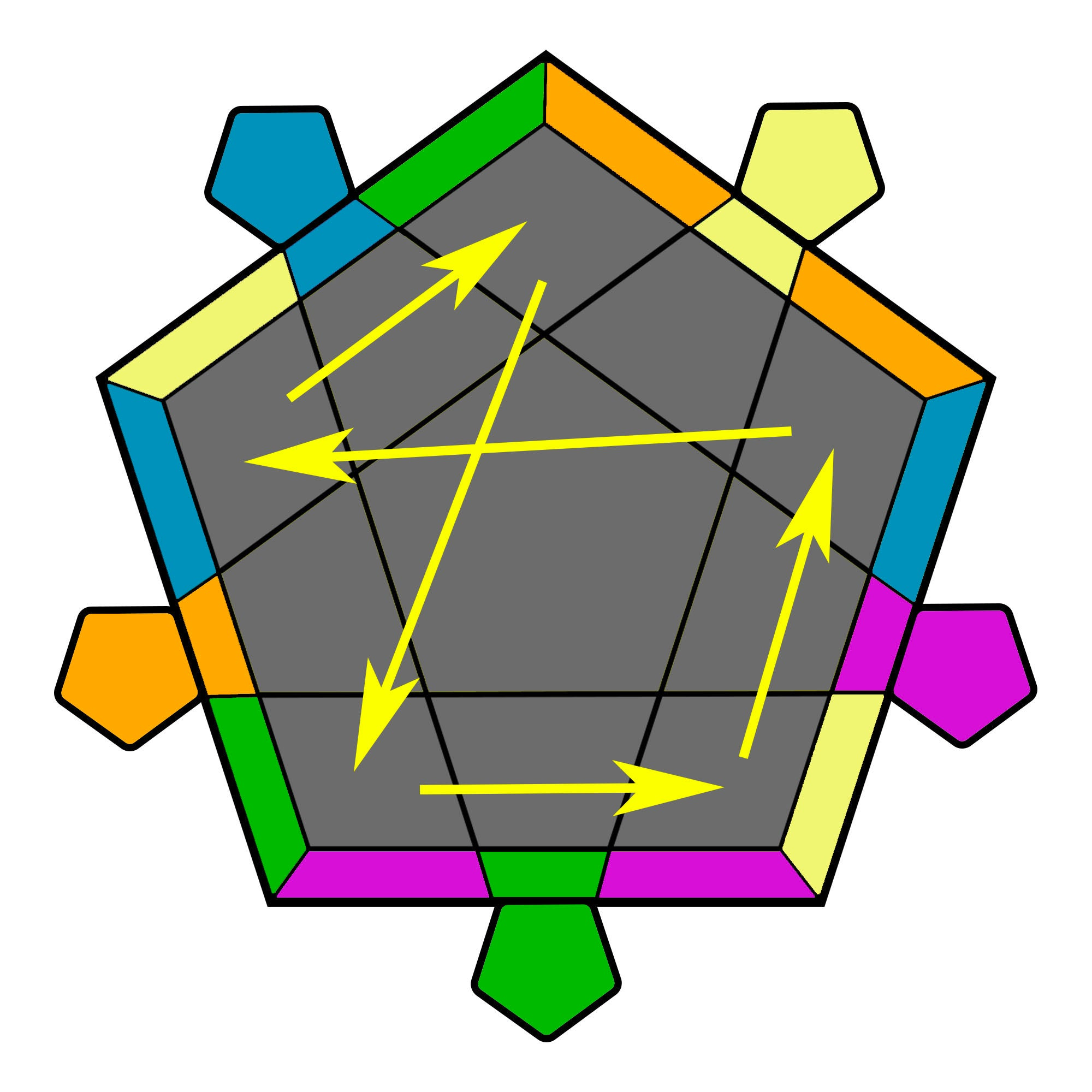(R' U2 R U') (R' U2 R U2') (R'U' R U2') (R' U R U2') (R' U R)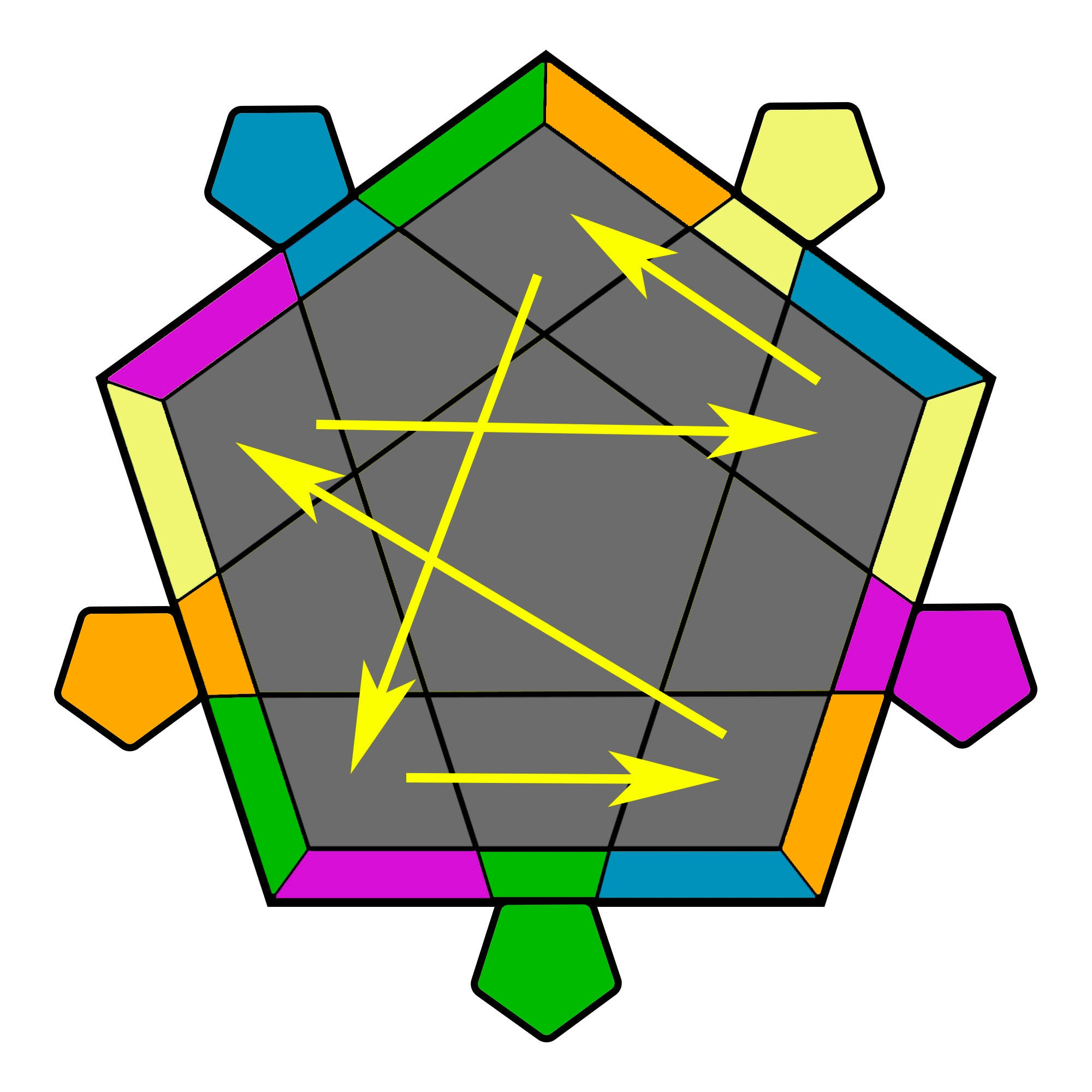(R2 U2' R2' U') (R2 U R2' U')(R2 U R2' U') (R2 U2' R2')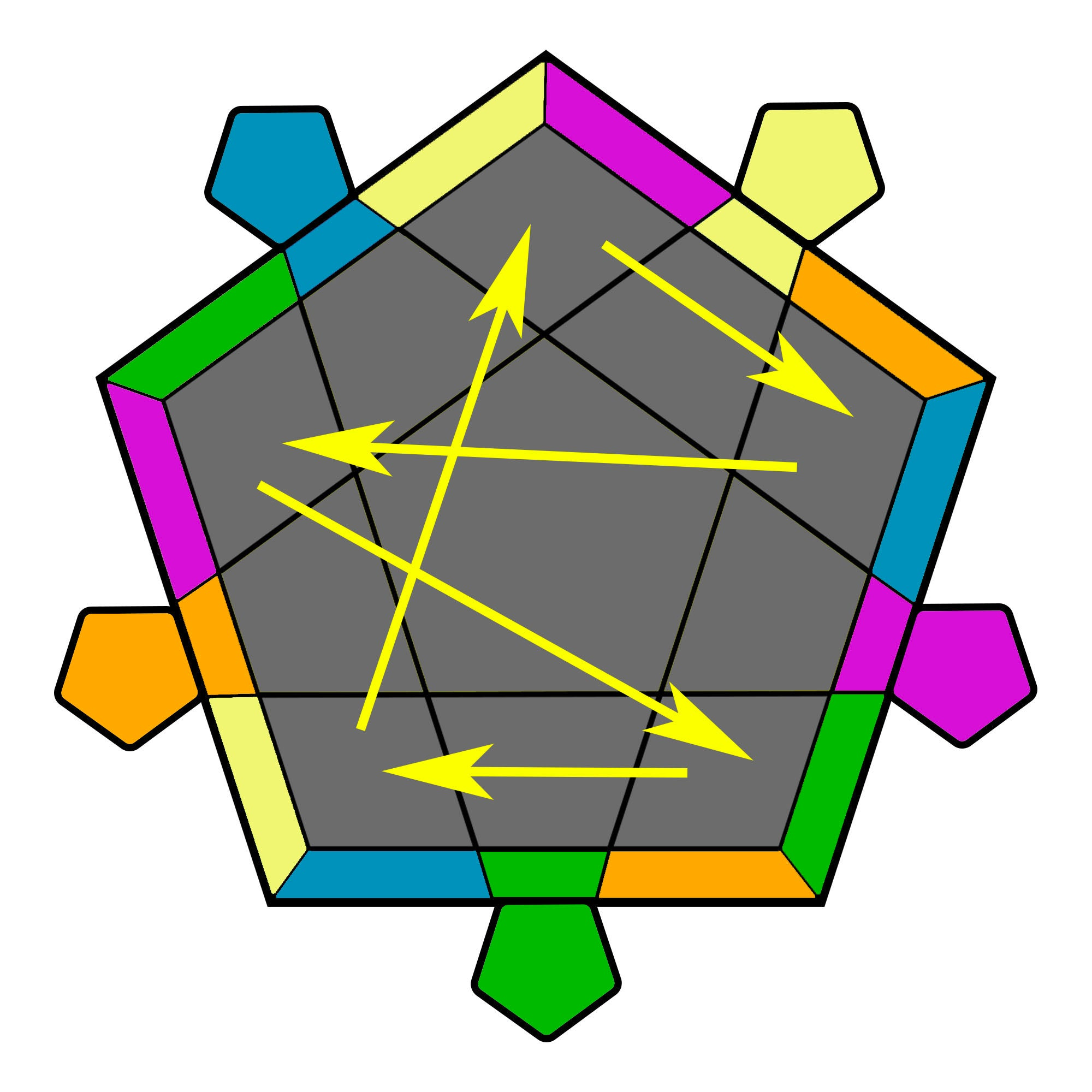(R2 U2 R2' U) (R2 U' R2' U)(R2 U' R2' U) (R2 U2 R2')